# 第 5 章 自然语言处理

## 5.1 如何用Python做情感分析？### 5.1.1 需求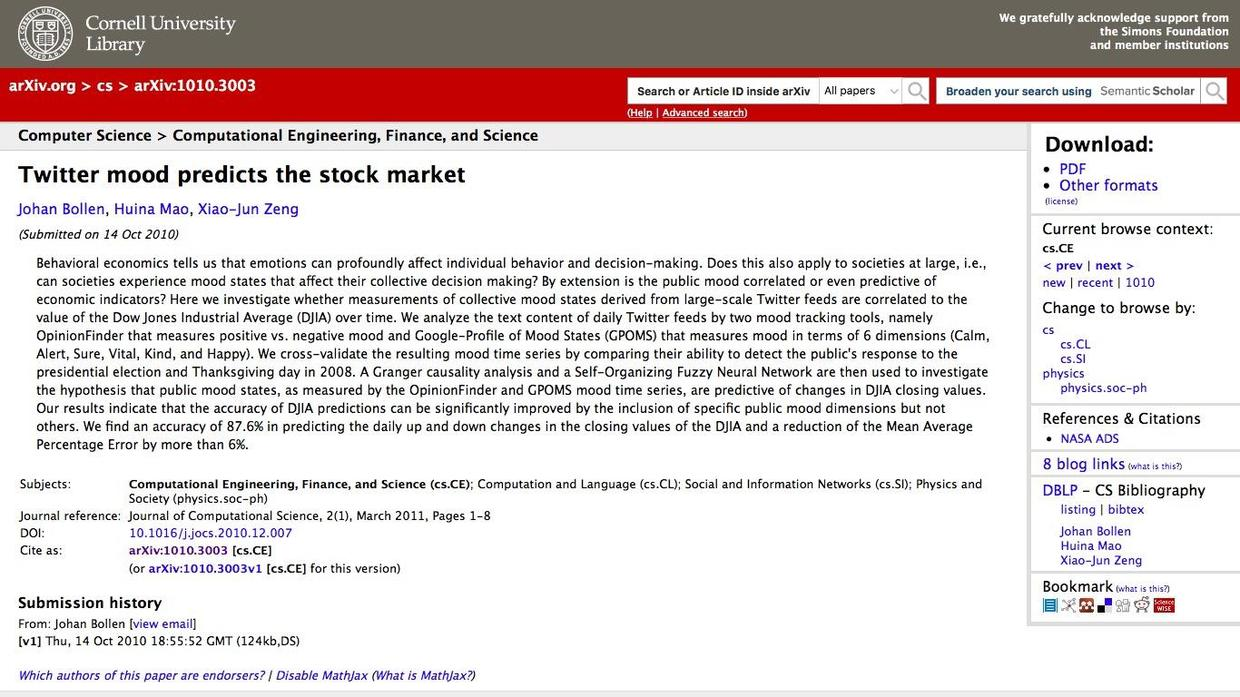### 5.1.2 安装

``````pip install snownlp
pip install -U textblob

``jupyter notebook``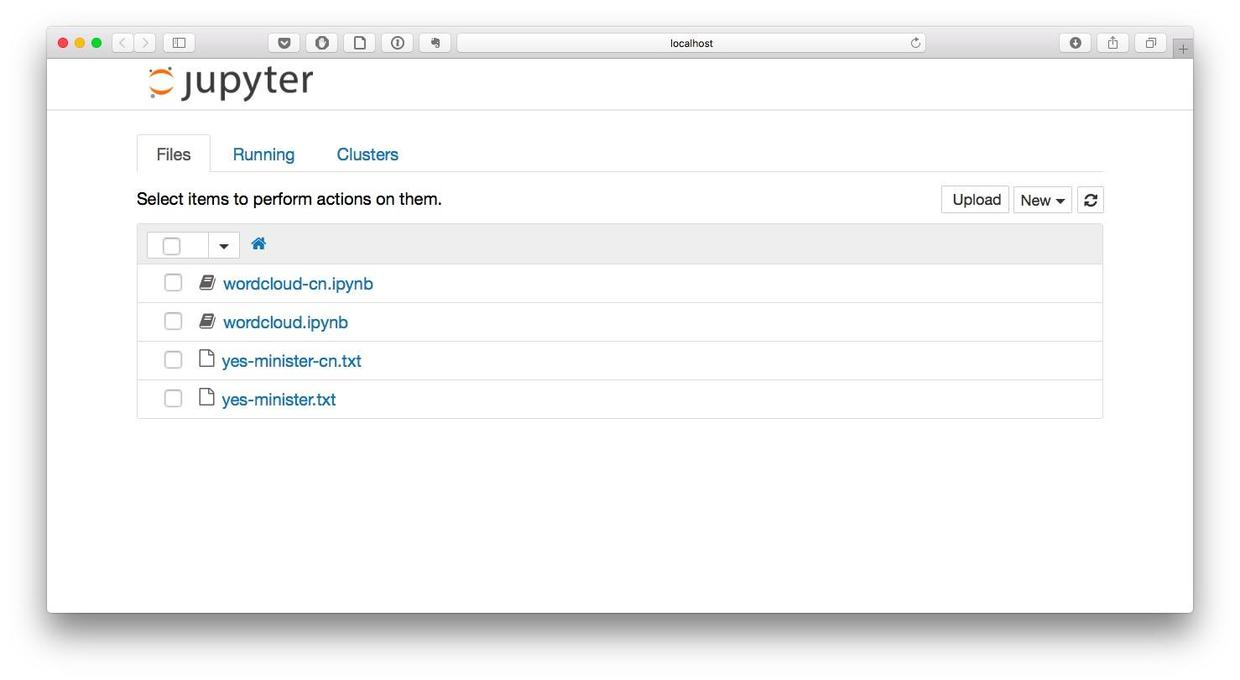### 5.1.3 英文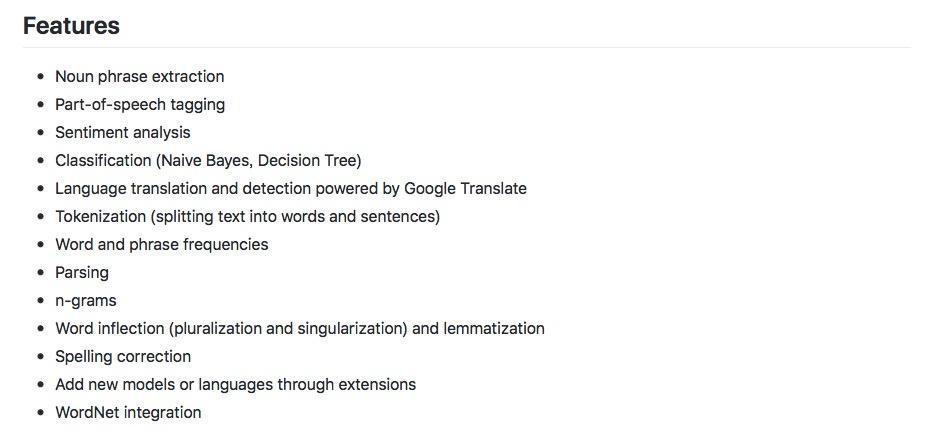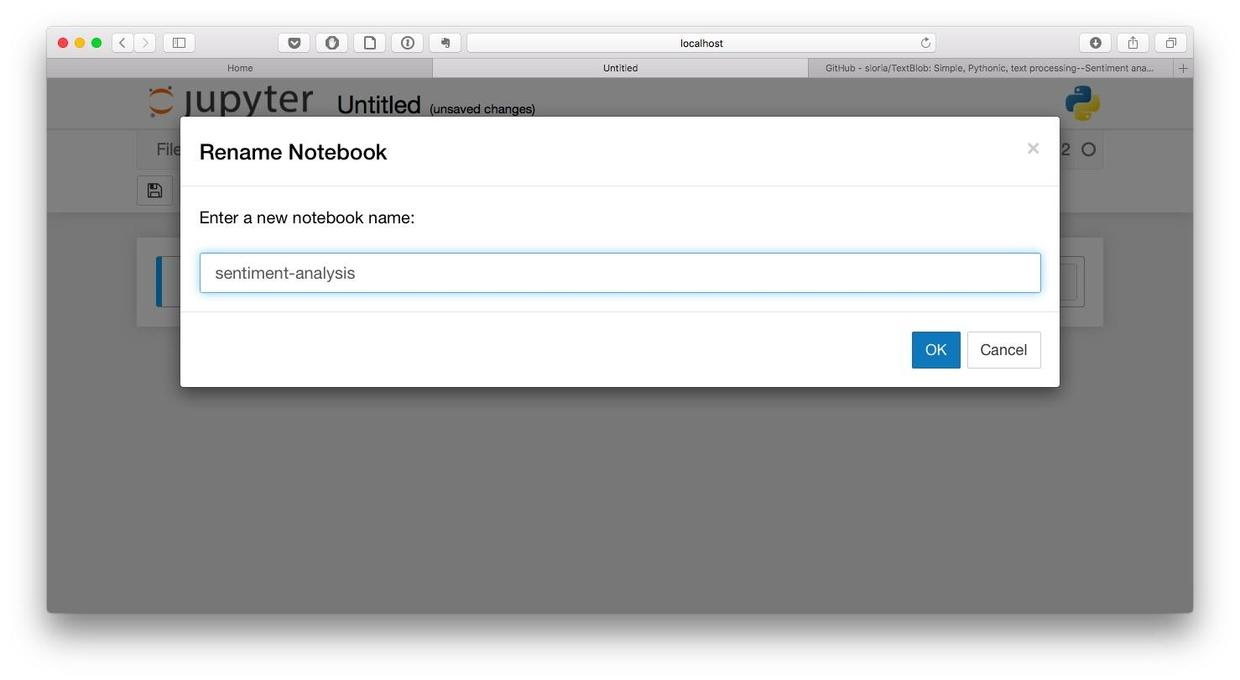``text = "I am happy today. I feel sad today."``

``````from textblob import TextBlob
blob = TextBlob(text)
blob``````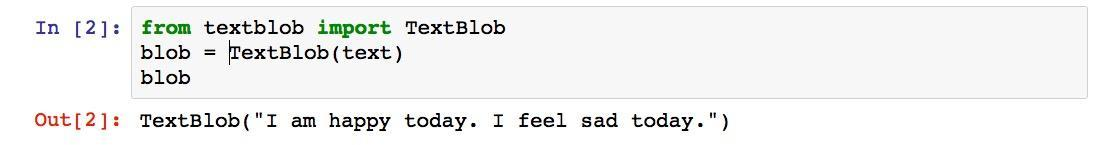``blob.sentences``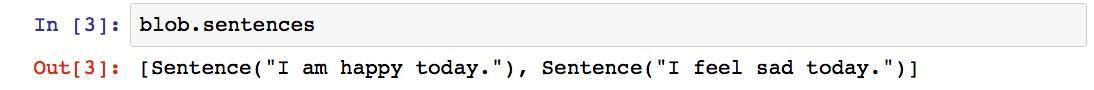``blob.sentences.sentiment``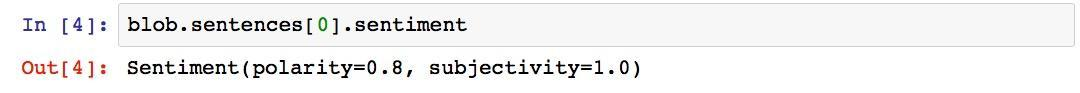``blob.sentences.sentiment``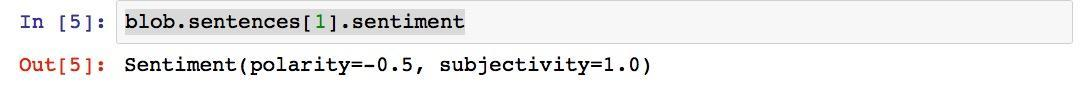“沮丧”对应的情感极性是负的0.5，没毛病！

``blob.sentiment``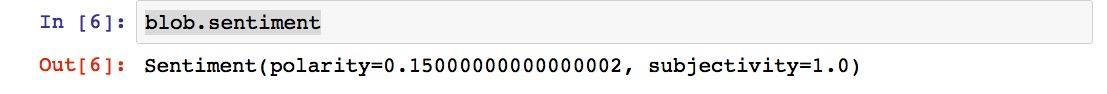### 5.1.4 中文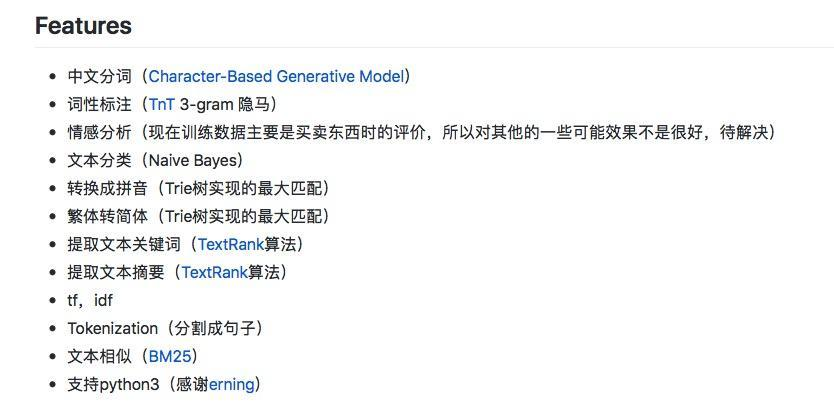``text = u"我今天很快乐。我今天很愤怒。"``

``````from snownlp import SnowNLP
s = SnowNLP(text)``````

``````for sentence in s.sentences:
print(sentence)``````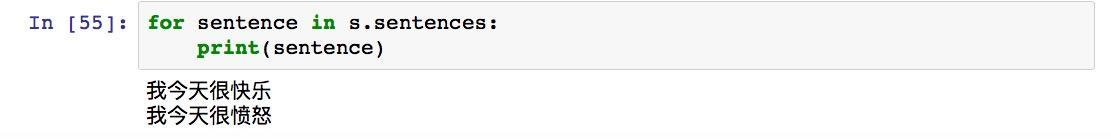``````s1 = SnowNLP(s.sentences)
s1.sentiments``````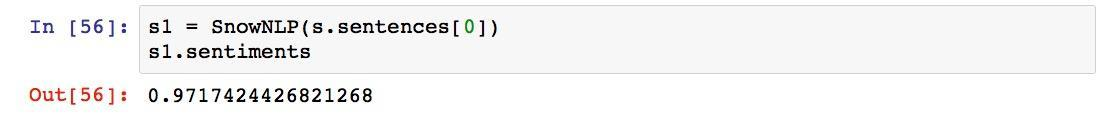``````s2 = SnowNLP(s.sentences)
s2.sentiments``````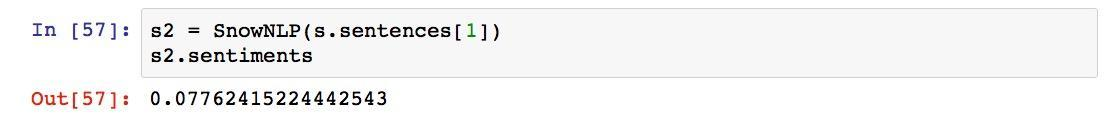## 5.2 如何用Python做舆情时间序列可视化？### 5.2.1 痛点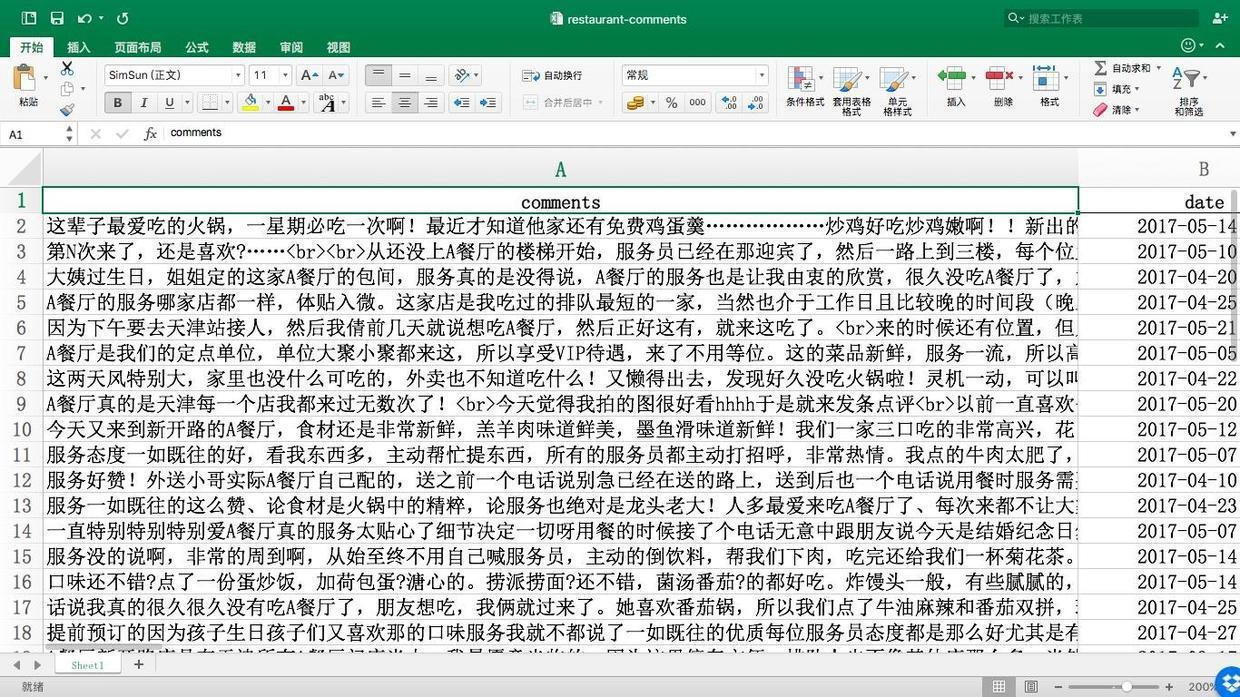### 5.2.2 序列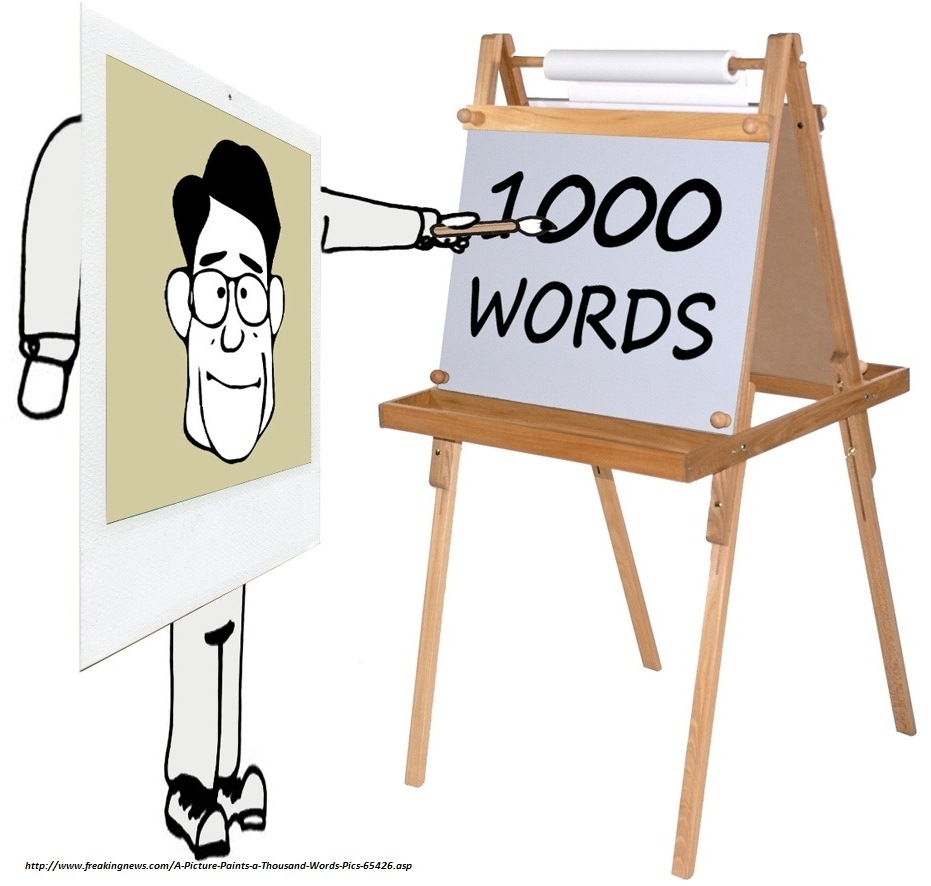### 5.2.3 准备``````pip install snownlp
pip install ggplot``````

``jupyter notebook``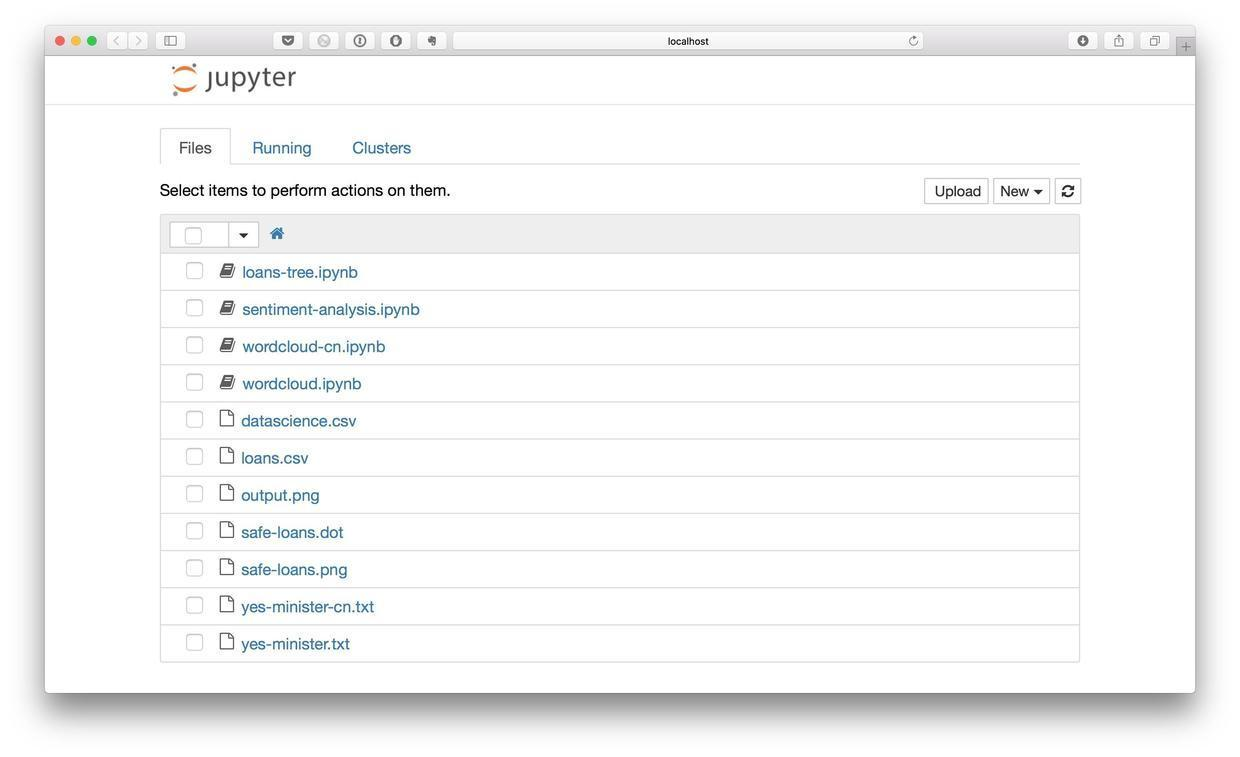### 5.2.4 代码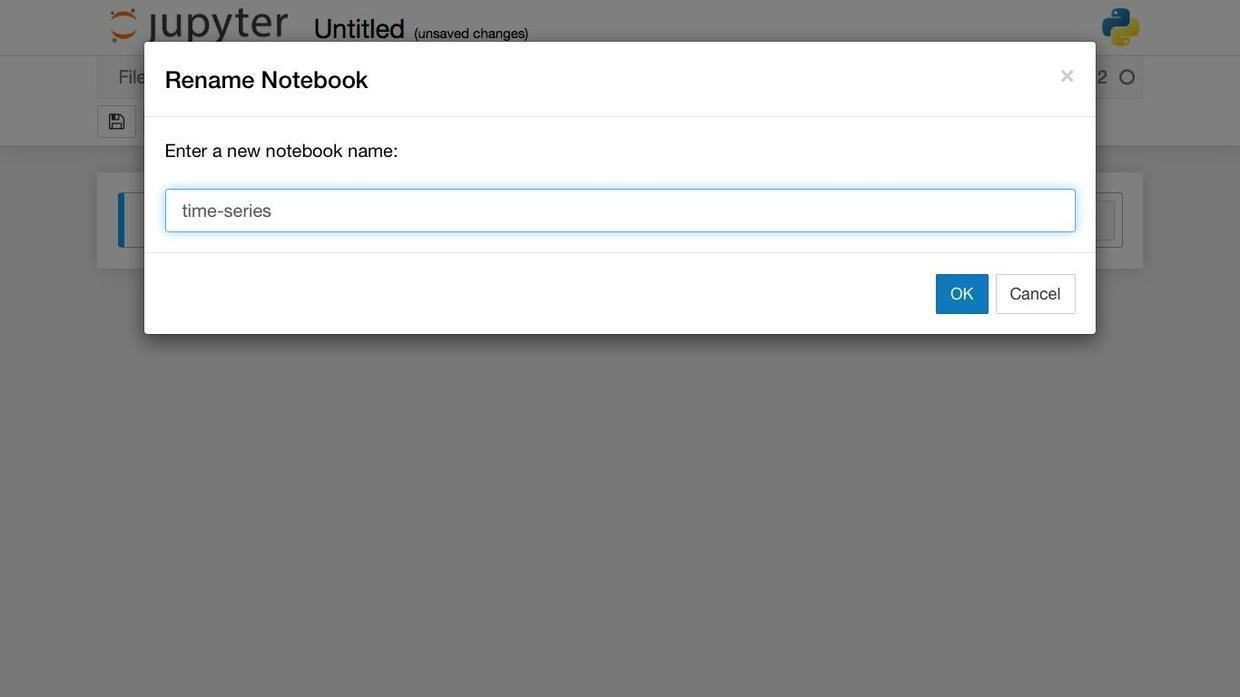``import pandas as pd``

``df = pd.read_excel("restaurant-comments.xlsx")``

``df.head()``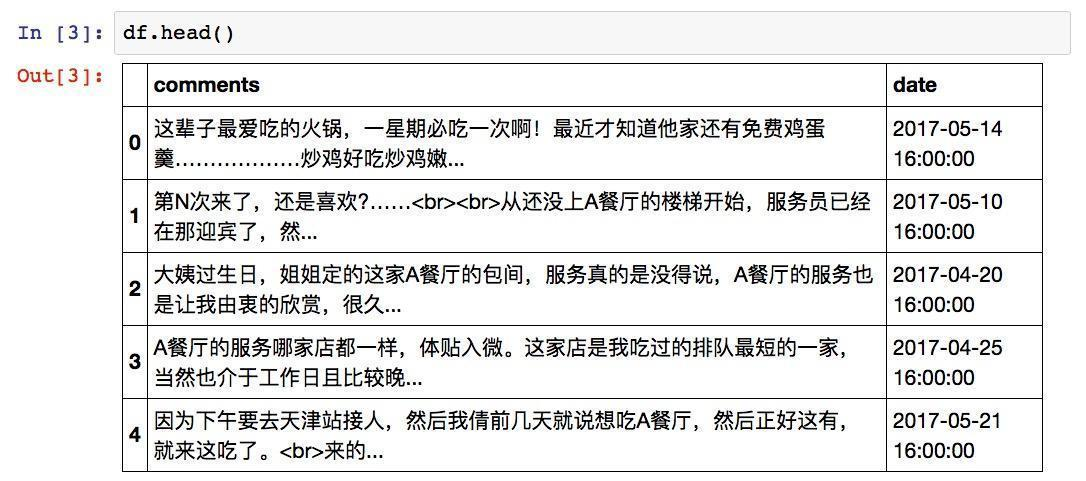``````from dateutil import parser
df["date"] = df.date.apply(parser.parse)``````

``text = df.comments.iloc``

``````from snownlp import SnowNLP
s = SnowNLP(text)``````

``s.sentiments``

``0.6331975099099649``

``````def get_sentiment_cn(text):
s = SnowNLP(text)
return s.sentiments``````

``df["sentiment"] = df.comments.apply(get_sentiment_cn)``

``df.head()``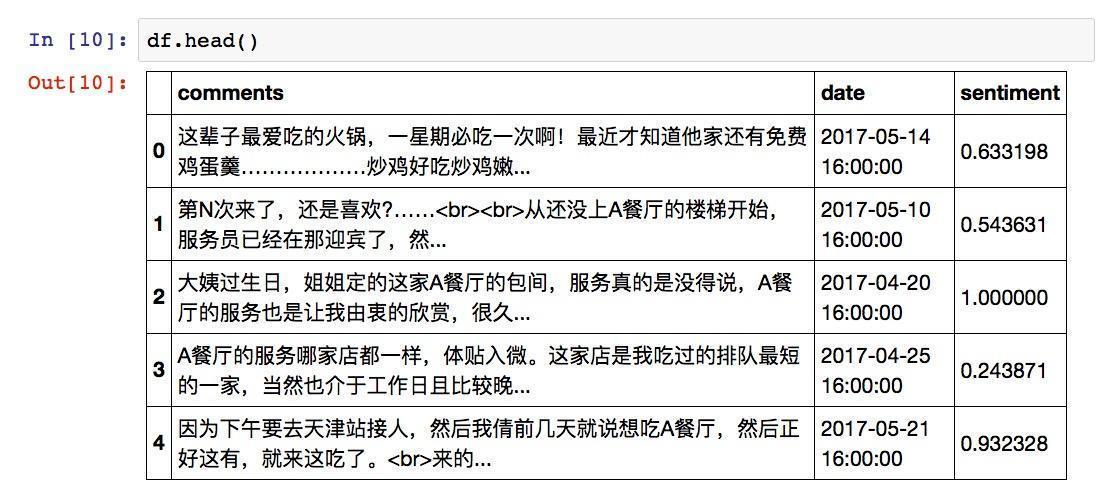``df.sentiment.mean()``

``0.7114015318571119``

``df.sentiment.median()``

``0.9563139038622388``

``````%pylab inline
from ggplot import *``````

``ggplot(aes(x="date", y="sentiment"), data=df) + geom_point() + geom_line(color = 'blue') + scale_x_date(labels = date_format("%Y-%m-%d"))``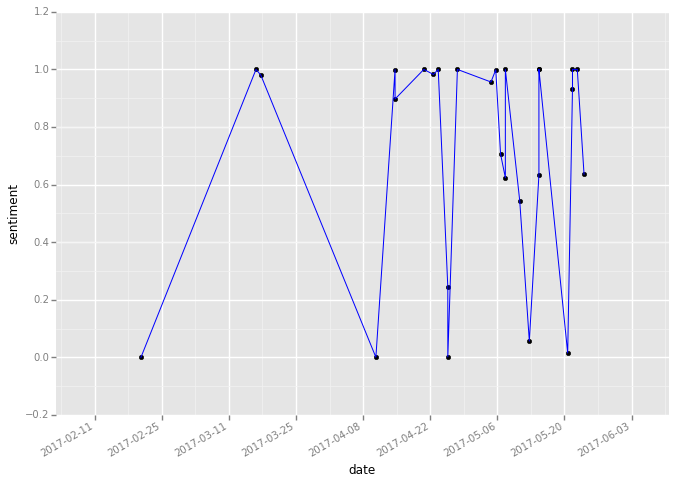``df.sort(['sentiment'])[:1]``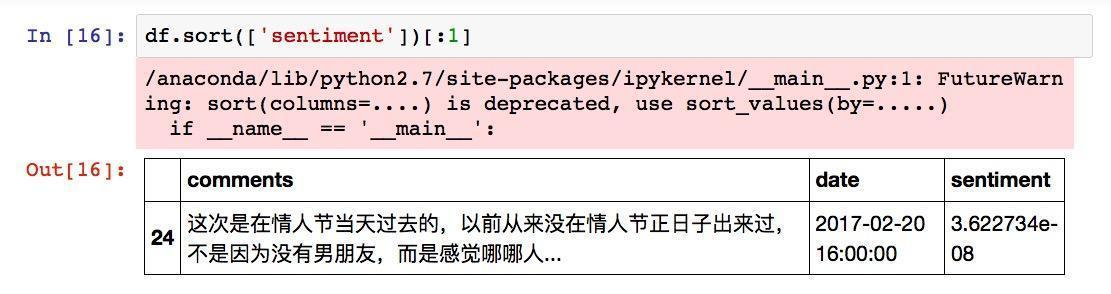``print(df.sort(['sentiment']).iloc.comments)``

## 5.3 如何用Python和R对故事情节做情绪分析？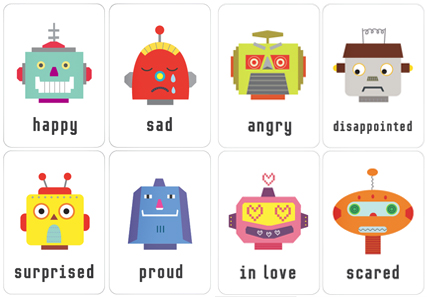### 5.3.1 烦恼

Netflix, Amazon和豆瓣等推荐引擎可以给你推荐影视作品。但是它们的推荐，只是把观众划分成了许多个圈子。你的数据，如果足够真实准确的话，可能刚好和某一个圈子的特性比较接近，于是就给你推荐这个圈子更喜欢的作品。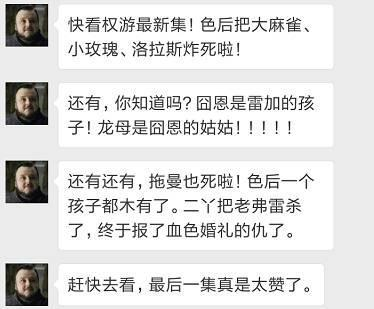You can’t have your cake and eat it too.

### 5.3.2 情绪

1. 愤怒(anger)
2. 期待(anticipation)
3. 厌恶(disgust)
4. 恐惧(fear)
5. 喜悦(joy)
7. 惊讶(surprise)
8. 信任(trust)

### 5.3.3 准备

#### 5.3.3.1 数据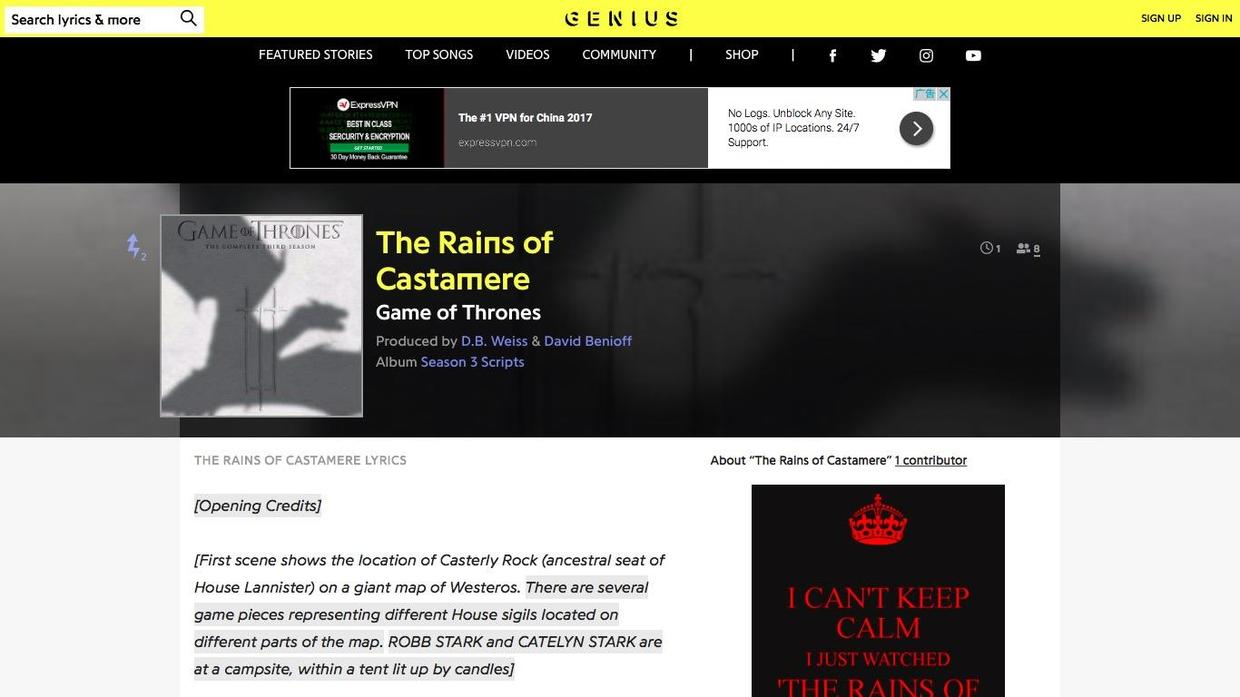#### 5.3.3.3 R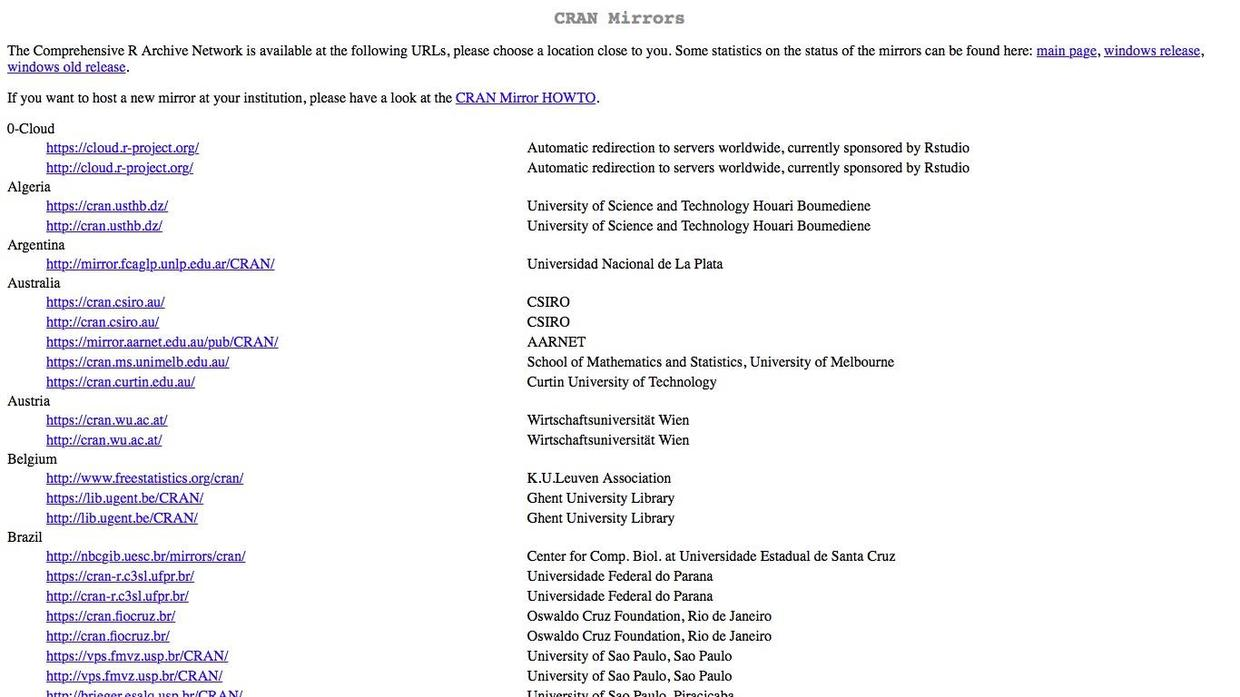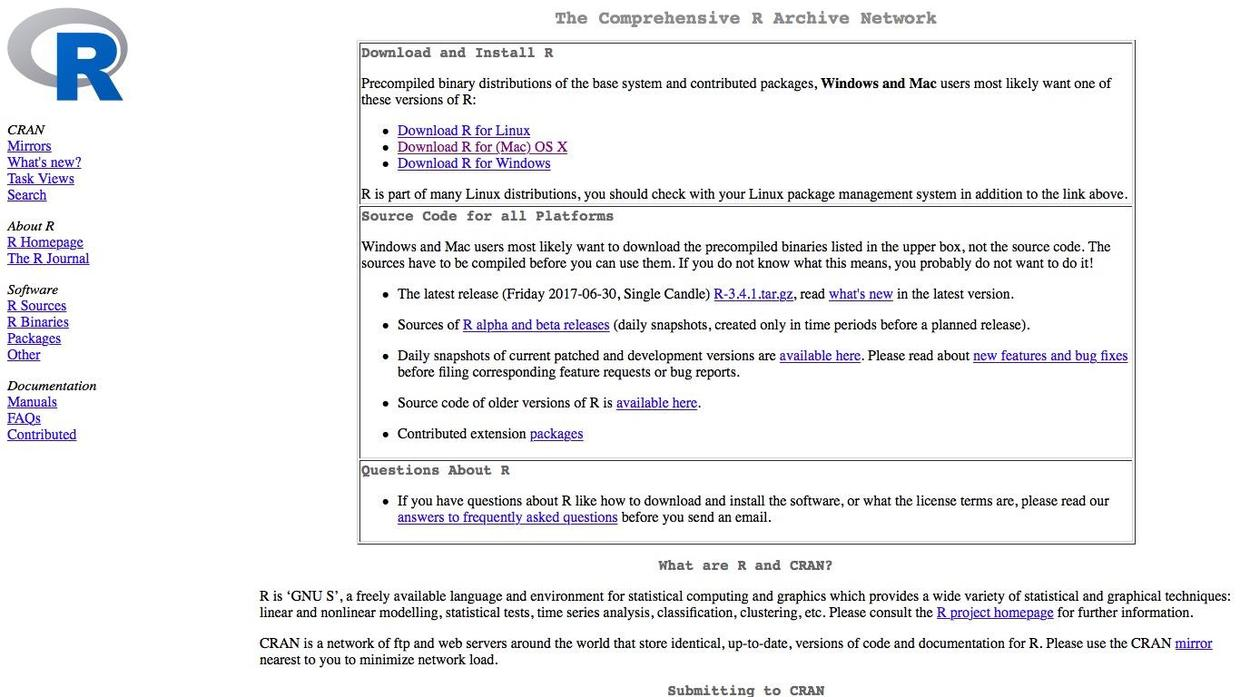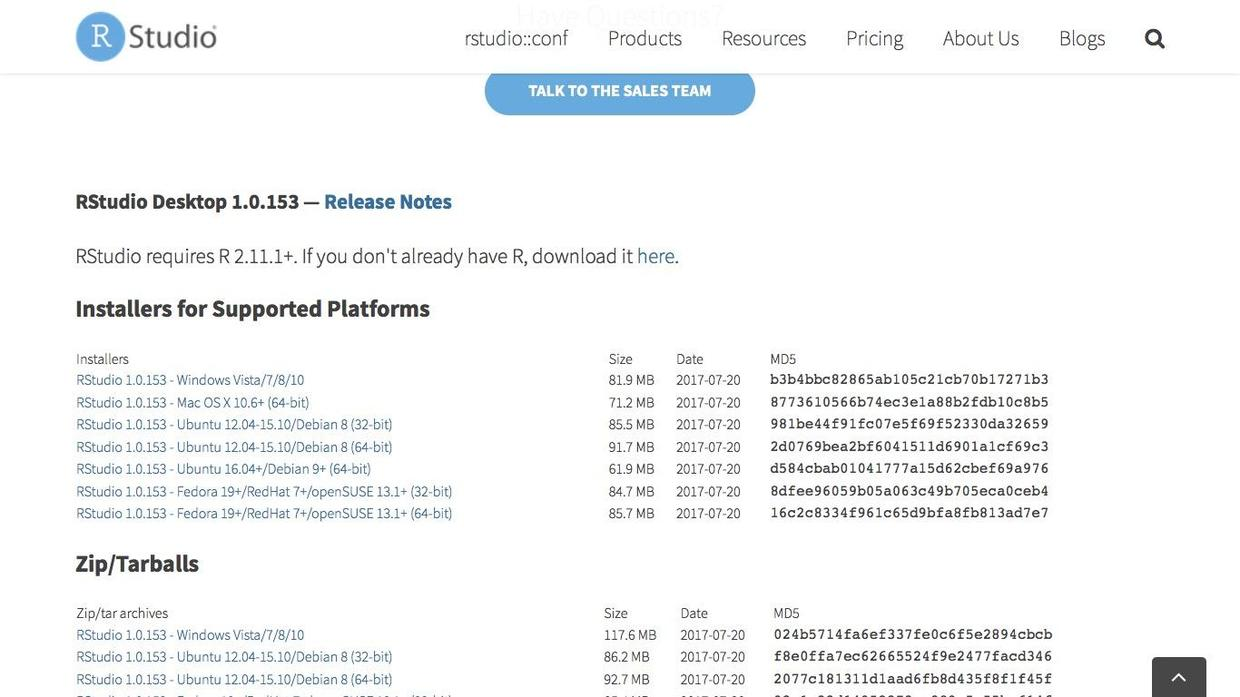### 5.3.4 清理

1. 把与剧情正文无关的内容去除；
2. 将数据转换成R可以直接做情绪分析的结构化数据格式。

``jupyter notebook``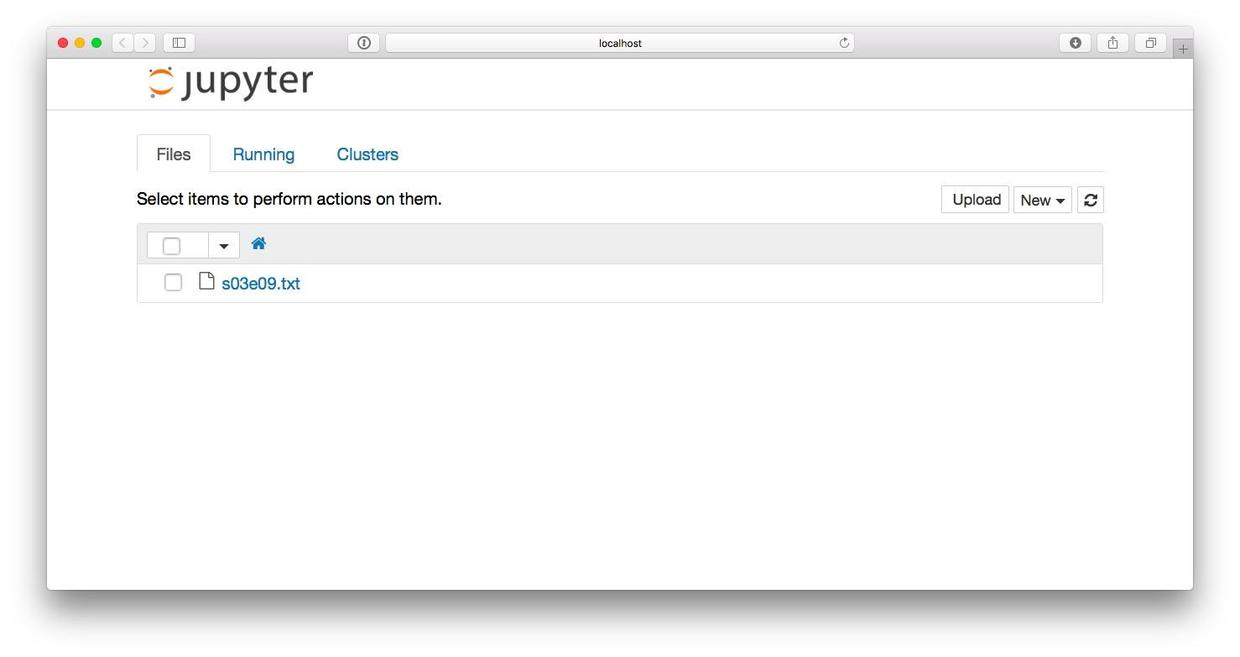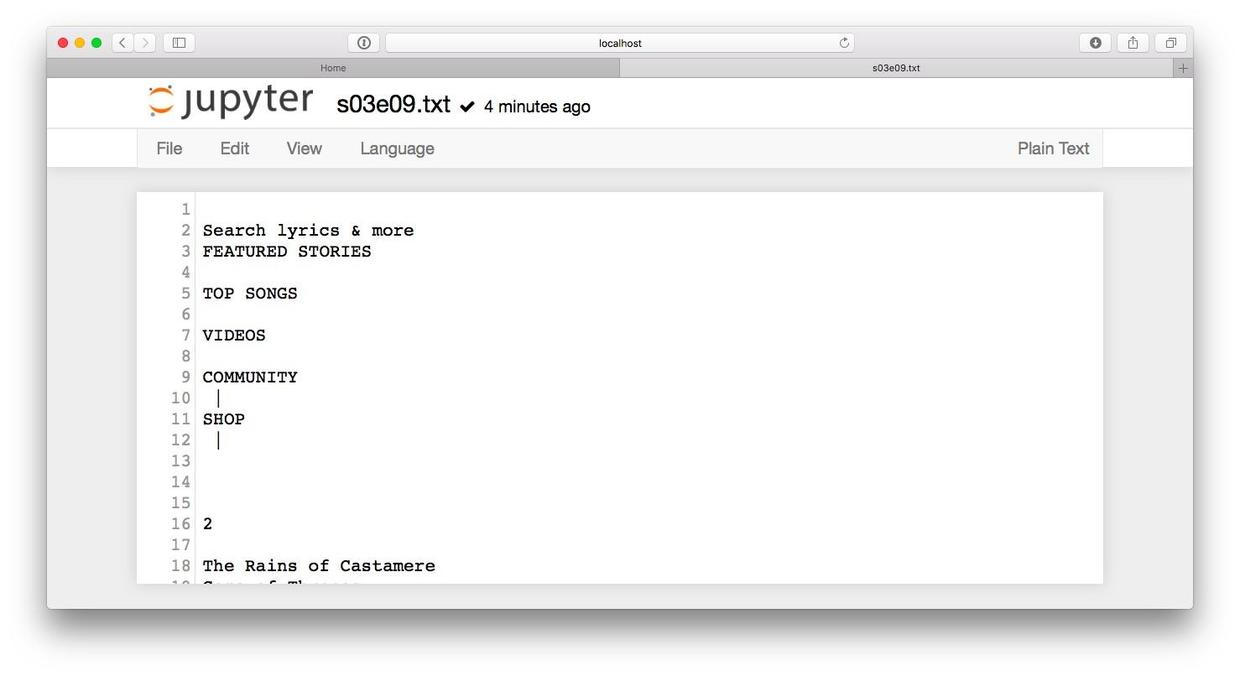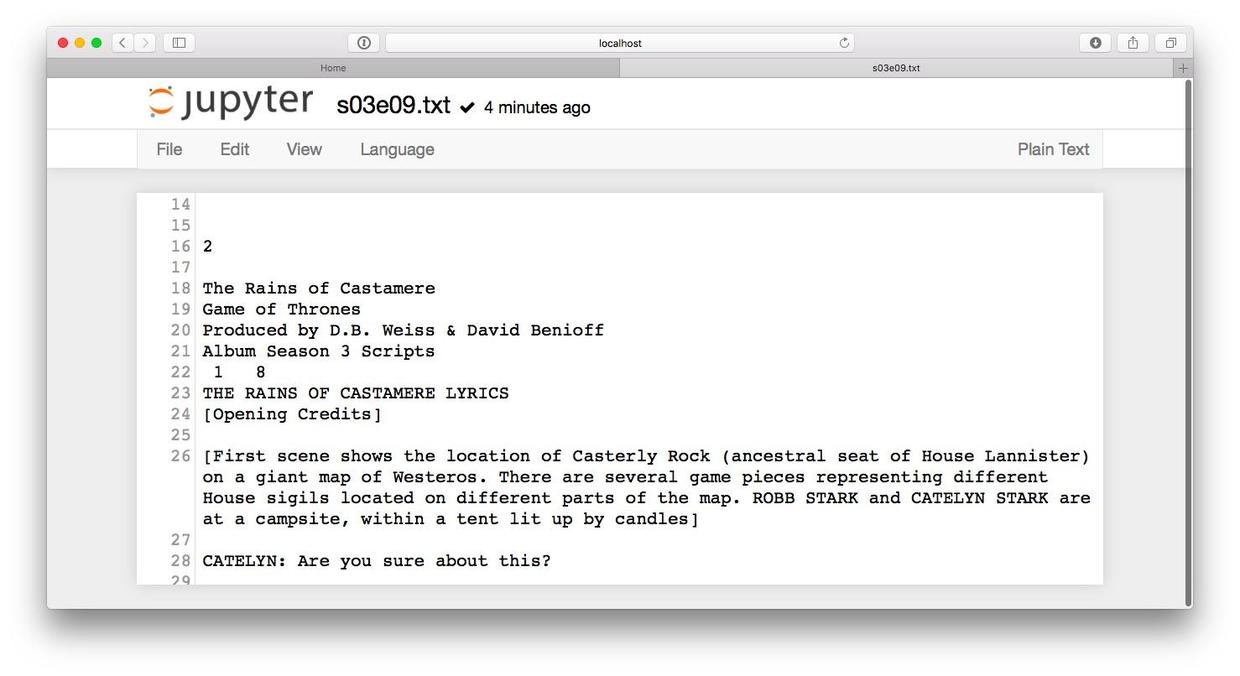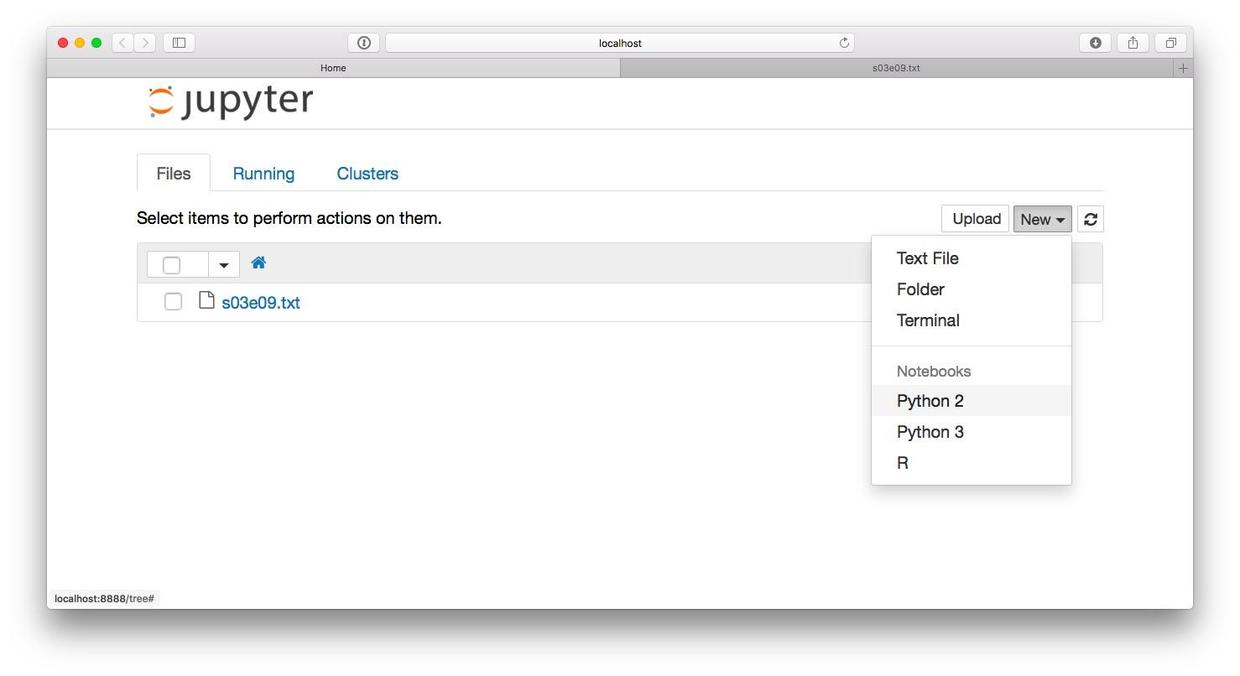``````import pandas as pd
import re``````

``````with open("s03e09.txt") as f:

``print(data)``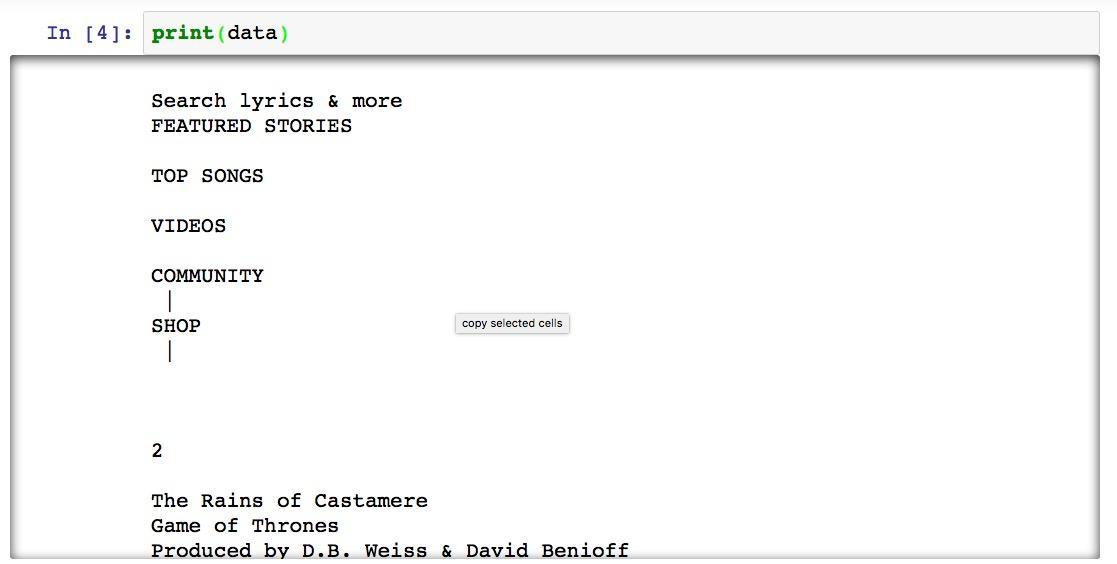``data = data.split('Opening Credits]')``

``print(data)``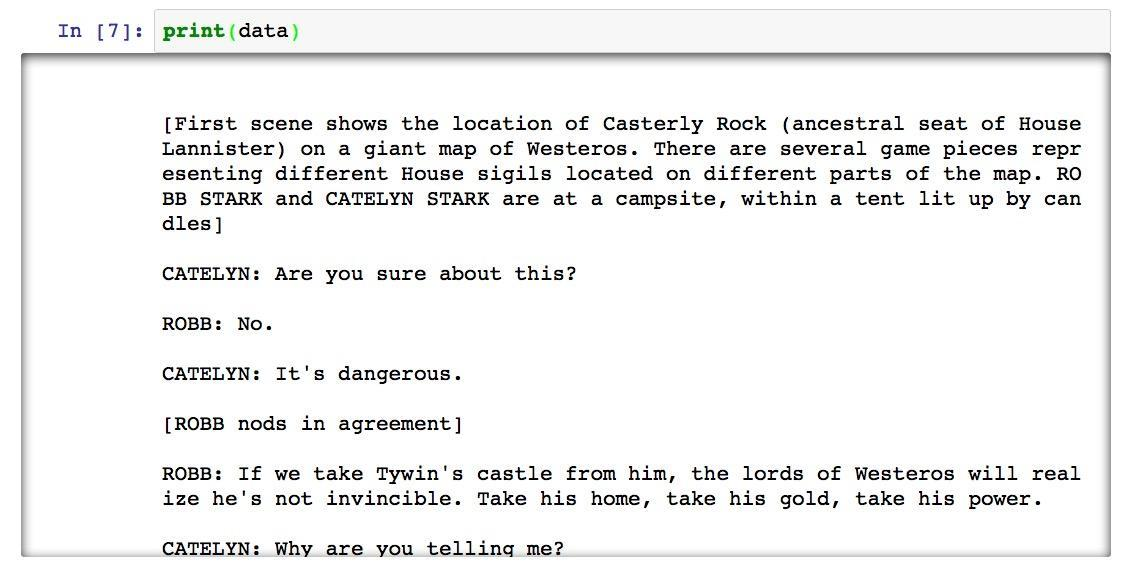``data = data.split('[End Credits')``

``print(data)``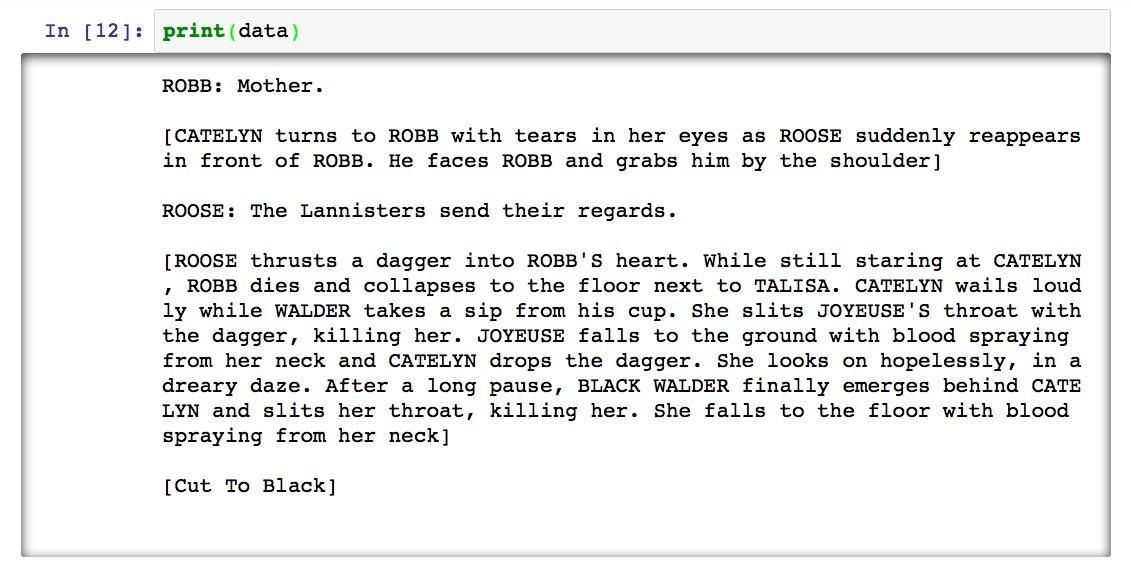``````regex = r"^\$\n"
subst = ""
data = re.sub(regex, subst, data, 0, re.MULTILINE)``````

``print(data)``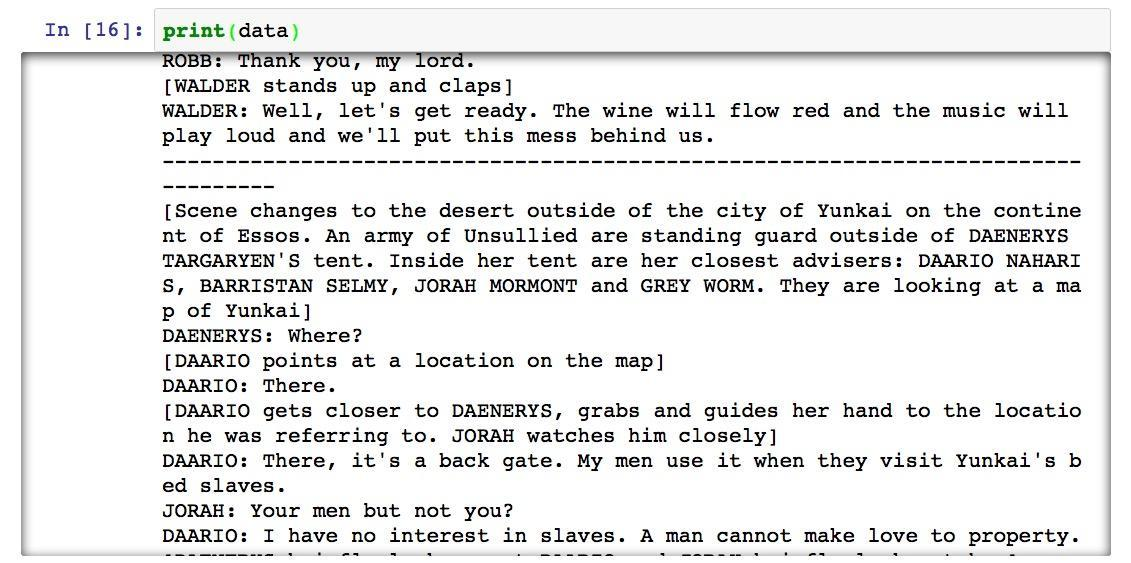``````regex = r"^-+\$\n"
subst = ""
data = re.sub(regex, subst, data, 0, re.MULTILINE)``````

``lines = data.split('\n')``

``````myrows = []
num = 1
for line in lines:
myrows.append([num, line])
num = num + 1``````

``myrows[:3]``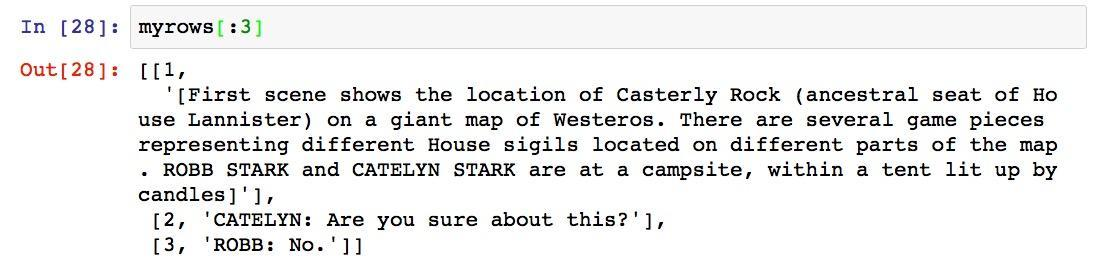``df = pd.DataFrame(myrows)``

``df.head()``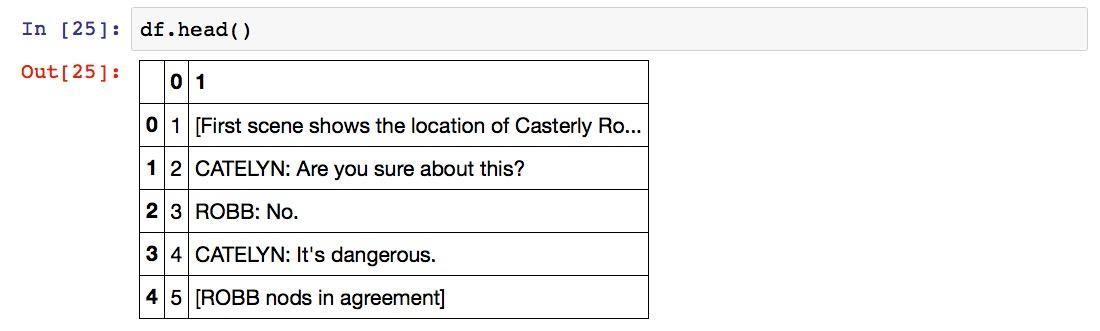``df.columns = ['line', 'text']``

``df.head()``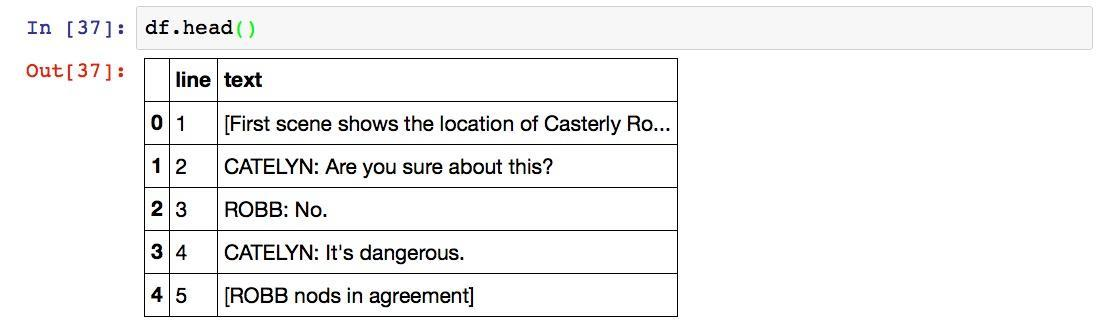``df.to_csv('data.csv', index=False)``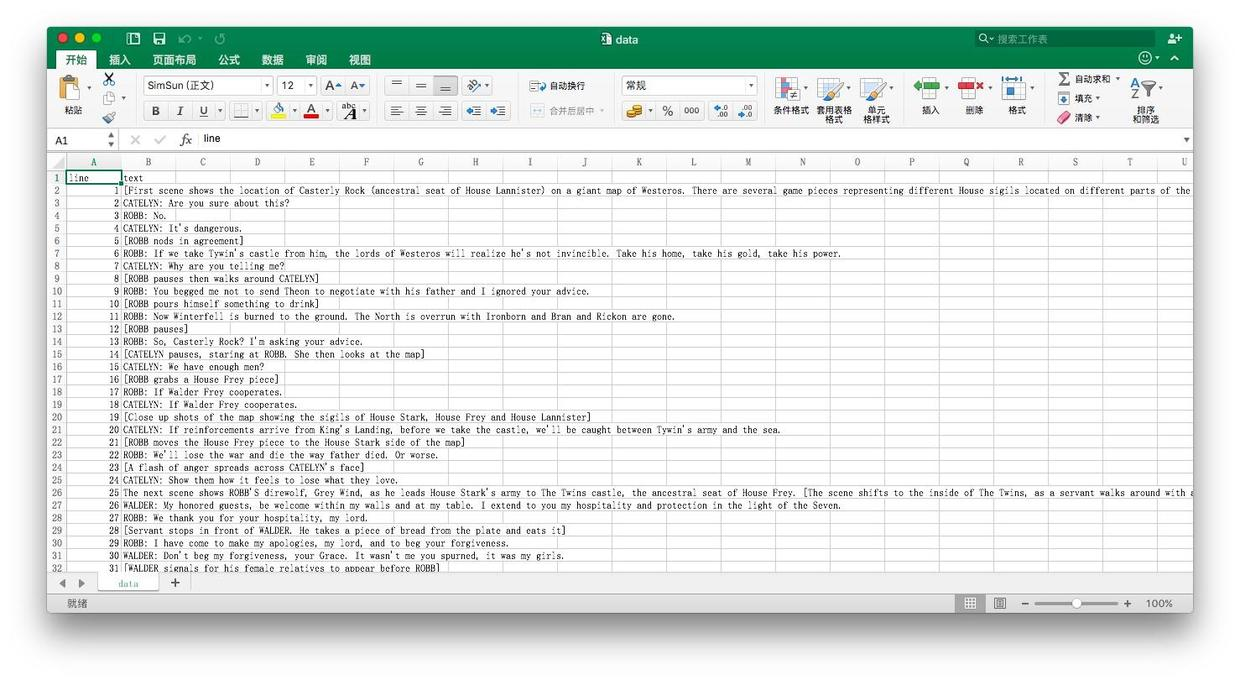### 5.3.5 分析

RStudio可以提供一个交互环境，帮我们执行R命令并即时反馈结果。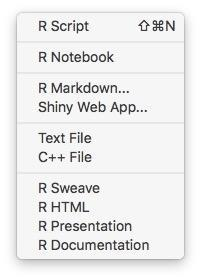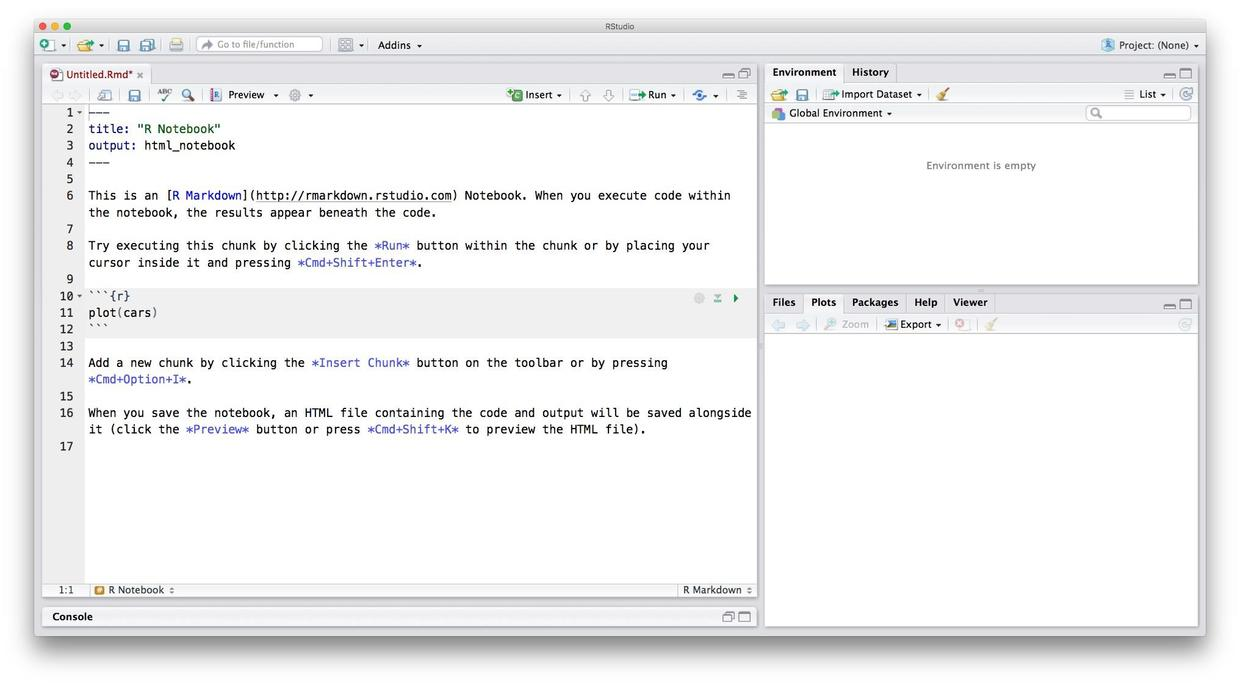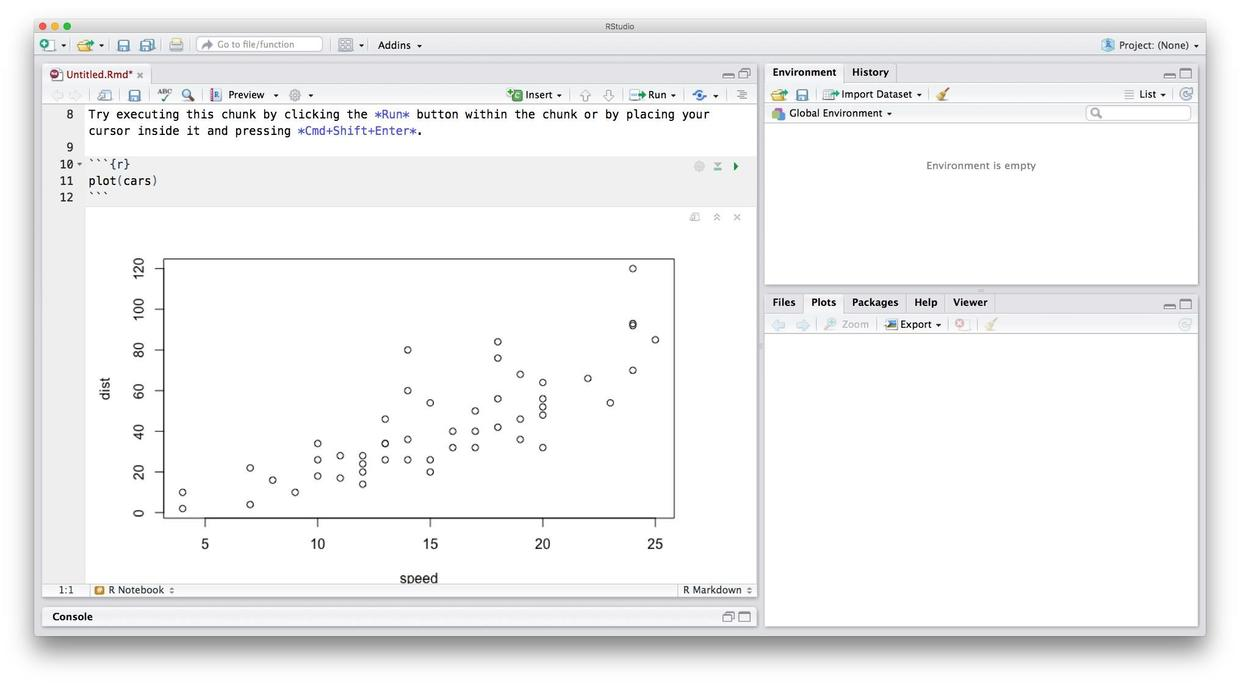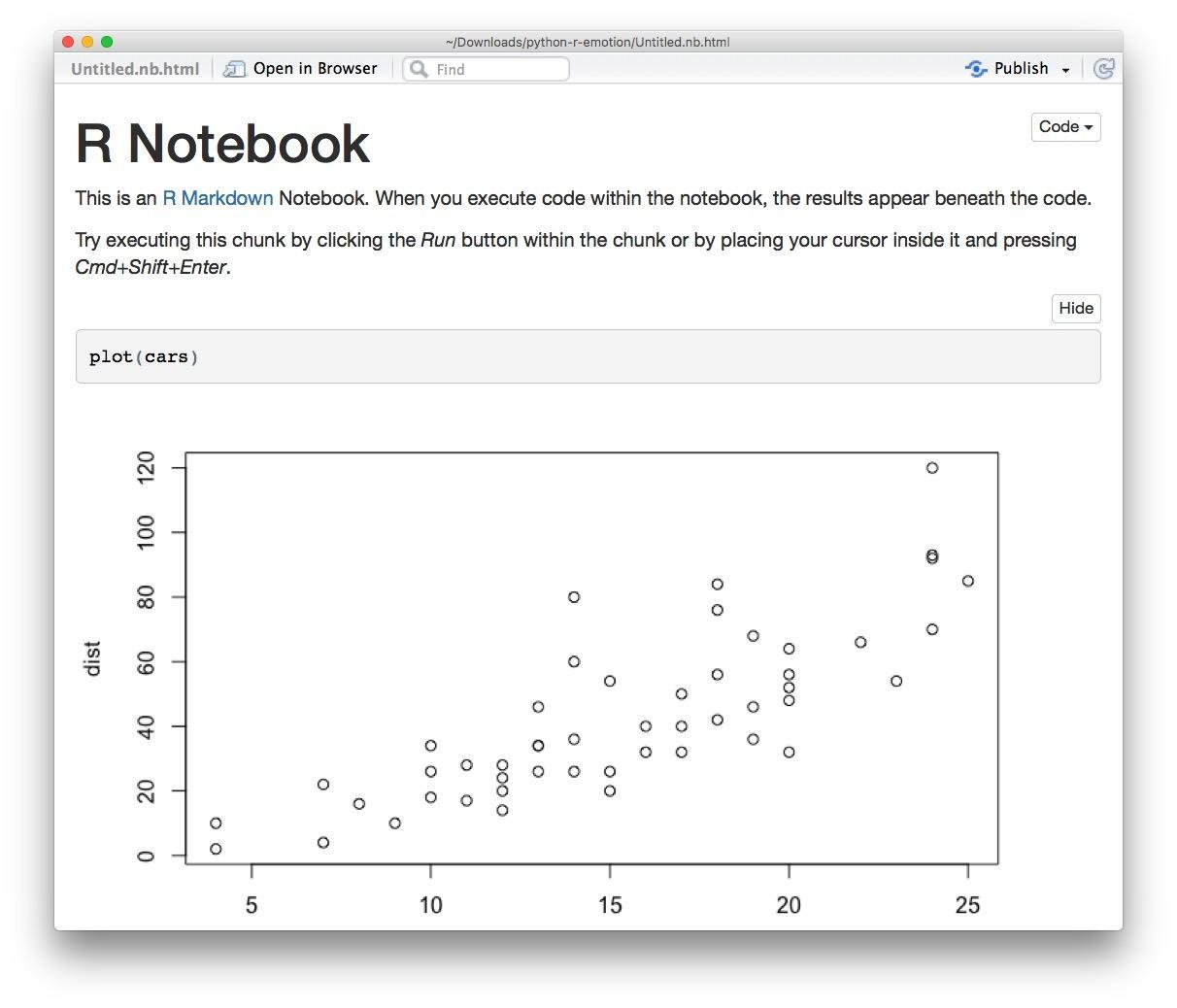RStudio为我们生成了HTML文件，我们的文字说明、代码和运行结果图文并茂呈现出来。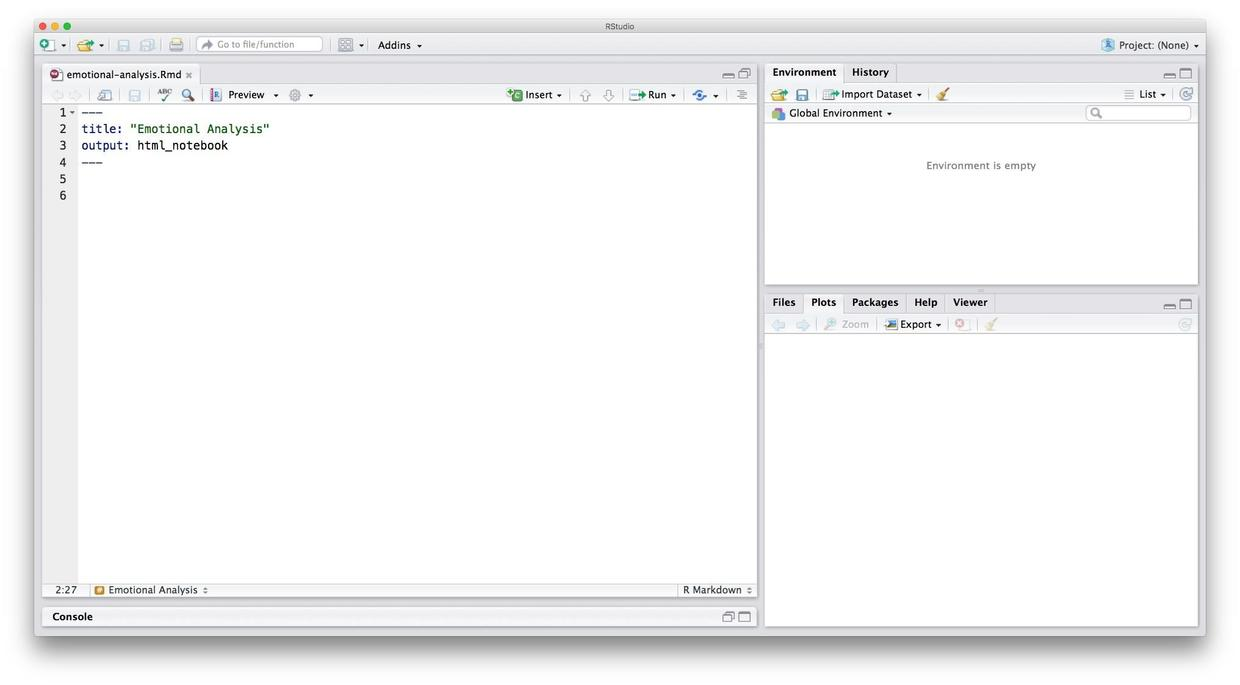``````setwd("~/Downloads/python-r-emotion/")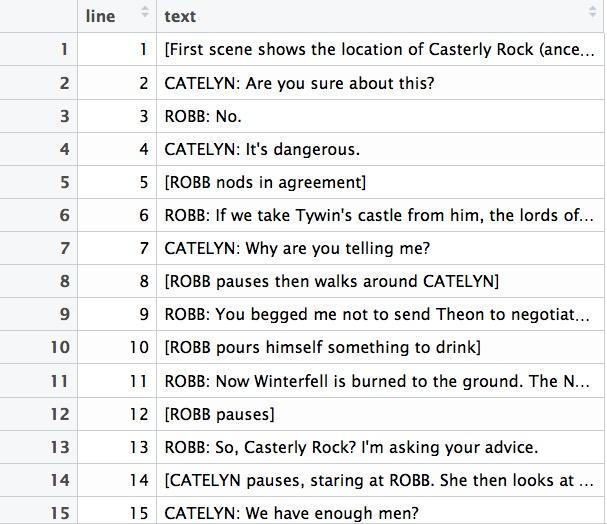``````install.packages("dplyr")
install.packages("tidytext")
install.packages("tidyr")
install.packages("ggplot2")``````

``````library(dplyr)
library(tidytext)
library(tidyr)
library(ggplot2)``````

``````tidy_script <- script %>%
unnest_tokens(word, text)
``````####     line     word

#### 1      1    first

#### 1.1    1    scene

#### 1.2    1    shows

#### 1.3    1      the

#### 1.4    1 location

#### 1.5    1       of
``````

``````tidy_script %>%
inner_join(get_sentiments("nrc")) %>%
arrange(line) %>%

``````#### Joining, by = "word"

####    line         word    sentiment

#### 1     1         rock     positive

#### 2     1    ancestral        trust

#### 3     1        giant         fear

#### 4     1 representing anticipation

#### 5     1        stark     negative

#### 6     1        stark        trust

#### 7     1        stark     negative

#### 8     1        stark        trust

#### 9     4    dangerous         fear

#### 10    4    dangerous     negative
``````

``````tidy_script %>%
inner_join(get_sentiments("nrc")) %>%
count(line, sentiment) %>%
arrange(line) %>%

``````#### Joining, by = "word"

#### # A tibble: 10 x 3

####     line    sentiment     n

####    <int>        <chr> <int>

####  1     1 anticipation     1

####  2     1         fear     1

####  3     1     negative     2

####  4     1     positive     1

####  5     1        trust     3

####  6     4         fear     1

####  7     4     negative     1

####  8     5     positive     1

####  9     5        trust     1

#### 10     6     positive     1
``````

``````tidy_script %>%
inner_join(get_sentiments("nrc")) %>%
count(line, sentiment) %>%
mutate(index = line %/% 5) %>%
arrange(index) %>%
``````#### Joining, by = "word"

#### # A tibble: 10 x 4

####     line    sentiment     n index

####    <int>        <chr> <int> <dbl>

####  1     1 anticipation     1     0

####  2     1         fear     1     0

####  3     1     negative     2     0

####  4     1     positive     1     0

####  5     1        trust     3     0

####  6     4         fear     1     0

####  7     4     negative     1     0

####  8     5     positive     1     1

####  9     5        trust     1     1

#### 10     6     positive     1     1
``````

``````tidy_script %>%
inner_join(get_sentiments("nrc")) %>%
count(line, sentiment) %>%
mutate(index = line %/% 5) %>%
ggplot(aes(x=index, y=n, color=sentiment)) %>%
+ geom_col()``````
``````#### Joining, by = "word"
``````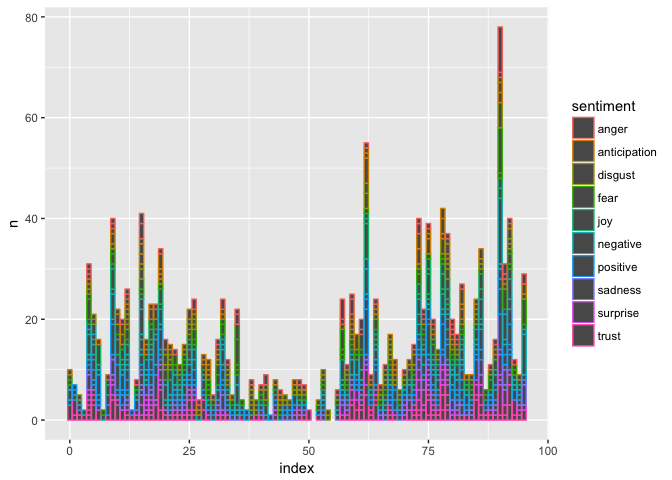``````tidy_script %>%
inner_join(get_sentiments("nrc")) %>%
count(line, sentiment) %>%
mutate(index = line %/% 5) %>%
ggplot(aes(x=index, y=n, color=sentiment)) %>%
+ geom_col() %>%
+ facet_wrap(~sentiment, ncol=3)``````
``````#### Joining, by = "word"
``````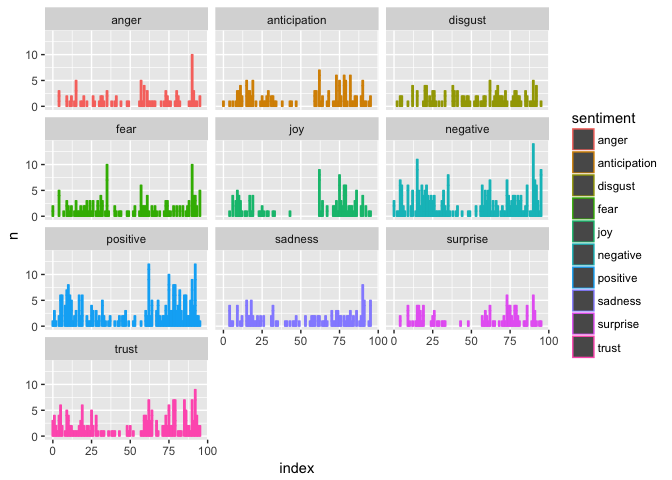``````tidy_script %>%
inner_join(get_sentiments("nrc")) %>%
filter(sentiment == "positive") %>%
count(word) %>%
arrange(desc(n)) %>%
``````#### Joining, by = "word"

#### # A tibble: 10 x 2

####        word     n

####       <chr> <int>

####  1     lord    13

####  2     good     9

####  3    guard     9

####  4 daughter     8

####  5 shoulder     7

####  6     love     6

####  7     main     6

####  8    quiet     6

####  9    bride     5

#### 10     king     5
``````

``````tidy_script %>%
inner_join(get_sentiments("nrc")) %>%
filter(sentiment == "negative") %>%
count(word) %>%
arrange(desc(n)) %>%
``````#### Joining, by = "word"

#### # A tibble: 10 x 2

####       word     n

####      <chr> <int>

####  1   stark    16

####  2     pig    14

####  3    lord    13

####  4    worm    12

####  5    kill    11

####  6   black     9

####  7  dagger     8

####  8    shot     8

####  9 killing     7

#### 10  afraid     4
``````

tidytext提供了默认的停用词表。我们先拿来试试看。这里使用的语句是`anti_join`，就可以把停用词先去除，再进行情绪词表连接。

``````tidy_script %>%
anti_join(stop_words) %>%
inner_join(get_sentiments("nrc")) %>%
filter(sentiment == "positive") %>%
count(word) %>%
arrange(desc(n)) %>%
``````#### Joining, by = "word"

#### Joining, by = "word"

#### # A tibble: 10 x 2

####        word     n

####       <chr> <int>

####  1     lord    13

####  2    guard     9

####  3 daughter     8

####  4 shoulder     7

####  5     love     6

####  6     main     6

####  7    quiet     6

####  8    bride     5

####  9     king     5

#### 10    music     5
``````

``````custom_stop_words <- bind_rows(stop_words,
data_frame(word = c("stark", "mother", "father", "daughter", "brother", "rock", "ground", "lord", "guard", "shoulder", "king", "main", "grace", "gate", "horse", "eagle", "servent"),
lexicon = c("custom")))``````

``````tidy_script %>%
anti_join(custom_stop_words) %>%
inner_join(get_sentiments("nrc")) %>%
filter(sentiment == "positive") %>%
count(word) %>%
arrange(desc(n)) %>%
``````#### Joining, by = "word"

#### Joining, by = "word"

#### # A tibble: 10 x 2

####           word     n

####          <chr> <int>

####  1        love     6

####  2       quiet     6

####  3       bride     5

####  4       music     5

####  5        rest     5

####  6     finally     4

####  7        food     3

####  8     forward     3

####  9        hope     3

#### 10 hospitality     3
``````

``````tidy_script %>%
anti_join(custom_stop_words) %>%
inner_join(get_sentiments("nrc")) %>%
filter(sentiment == "negative") %>%
count(word) %>%
arrange(desc(n)) %>%
``````#### Joining, by = "word"

#### Joining, by = "word"

#### # A tibble: 10 x 2

####       word     n

####      <chr> <int>

####  1     pig    14

####  2    worm    12

####  3    kill    11

####  4   black     9

####  5  dagger     8

####  6    shot     8

####  7 killing     7

####  8  afraid     4

####  9    fear     4

#### 10   leave     4
``````

``````tidy_script %>%
anti_join(custom_stop_words) %>%
inner_join(get_sentiments("nrc")) %>%
filter(sentiment != "negative" & sentiment != "positive") %>%
count(line, sentiment) %>%
mutate(index = line %/% 5) %>%
ggplot(aes(x=index, y=n, color=sentiment)) %>%
+ geom_col() %>%
+ facet_wrap(~sentiment, ncol=3)``````
``````#### Joining, by = "word"

#### Joining, by = "word"
``````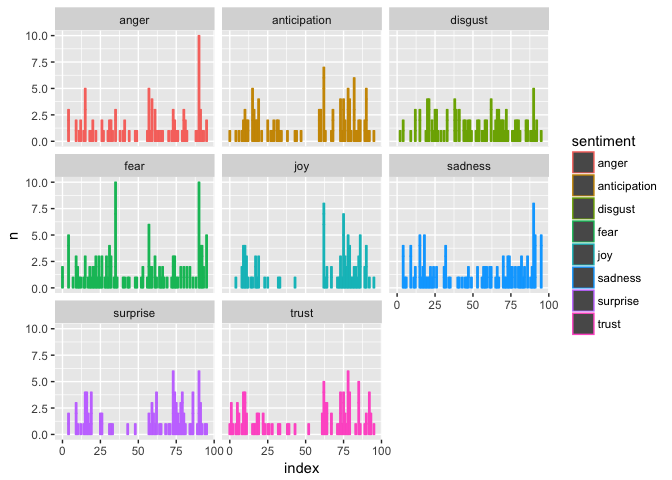### 5.3.6 收获

1. 如何用Python对网络摘取的文本做处理，从中找出正文，并且去掉空行等内容；
2. 如何用数据框对数据进行存储、表示与格式转换，在Python和R中交换数据；
3. 如何安装和使用RStudio环境，用R Notebook做交互式编程；
4. 如何利用tidytext方式来处理情感分析与情绪分析；
5. 如何设置自己的停用词表；
6. 如何用ggplot绘制多维度切面图形。

## 5.4 如何用Python提取中文关键词？### 5.4.2 环境

#### 5.4.2.1 Python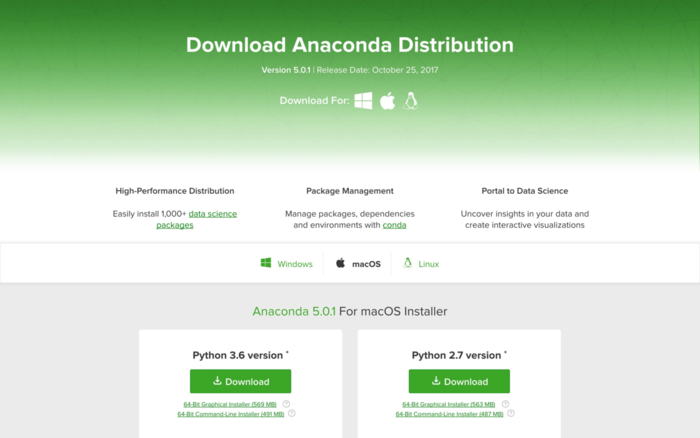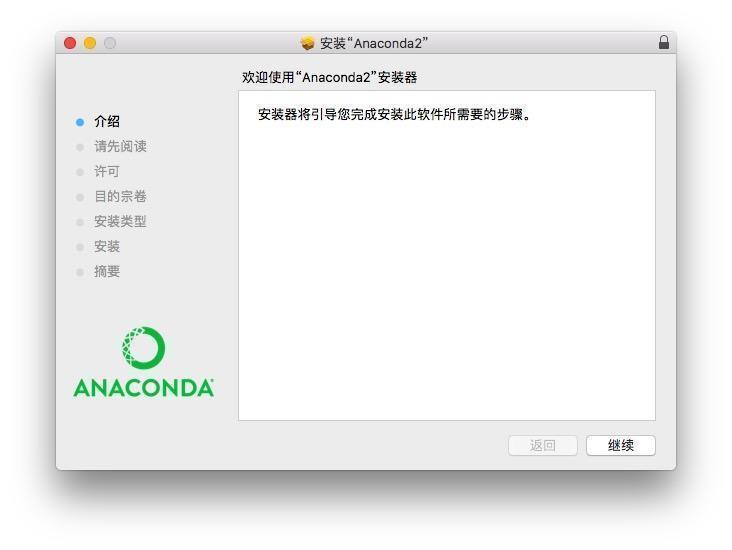#### 5.4.2.2 样例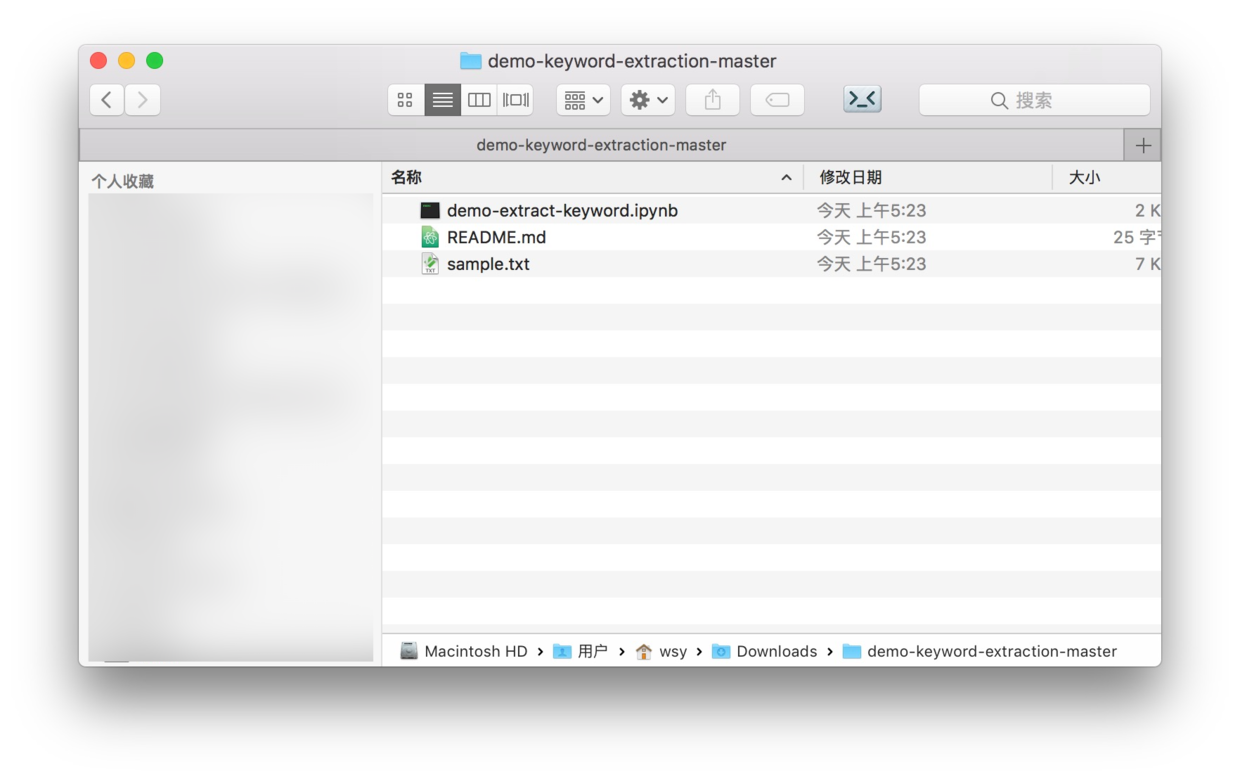#### 5.4.2.3 结巴分词

``pip install jieba``

``jupyter notebook``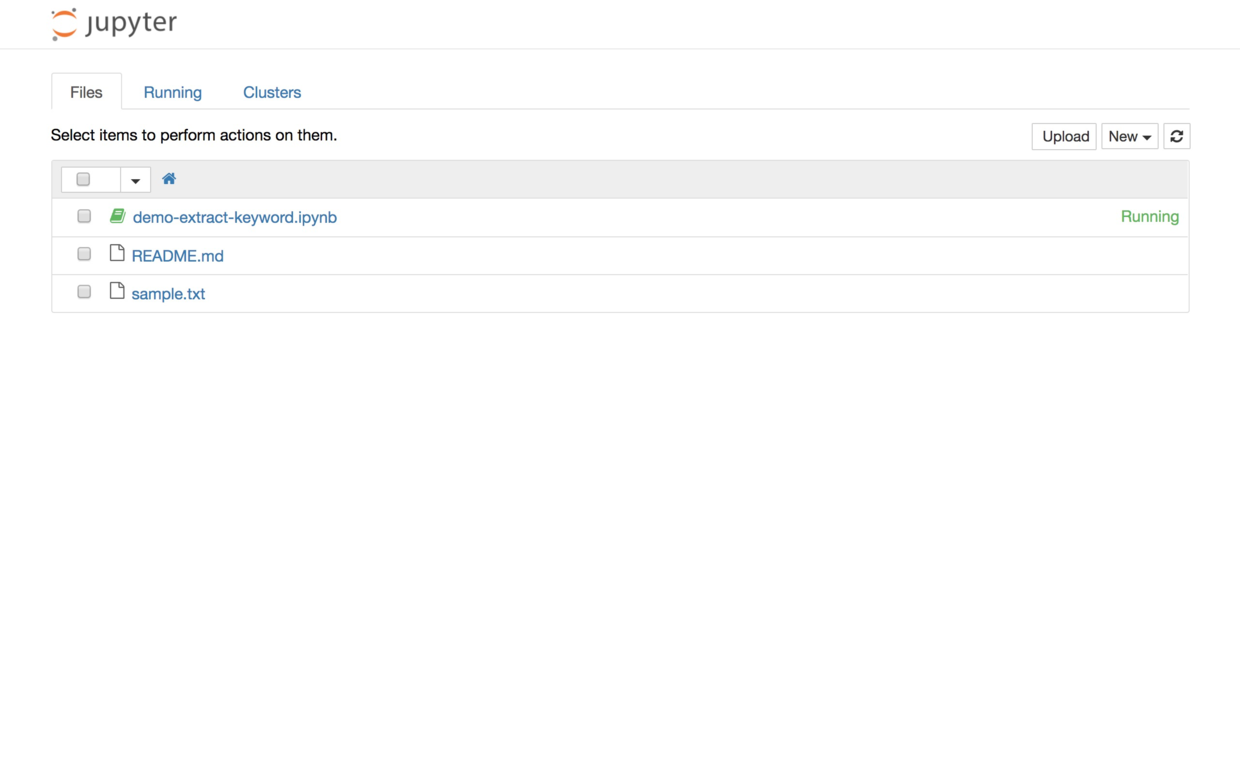### 5.4.3 数据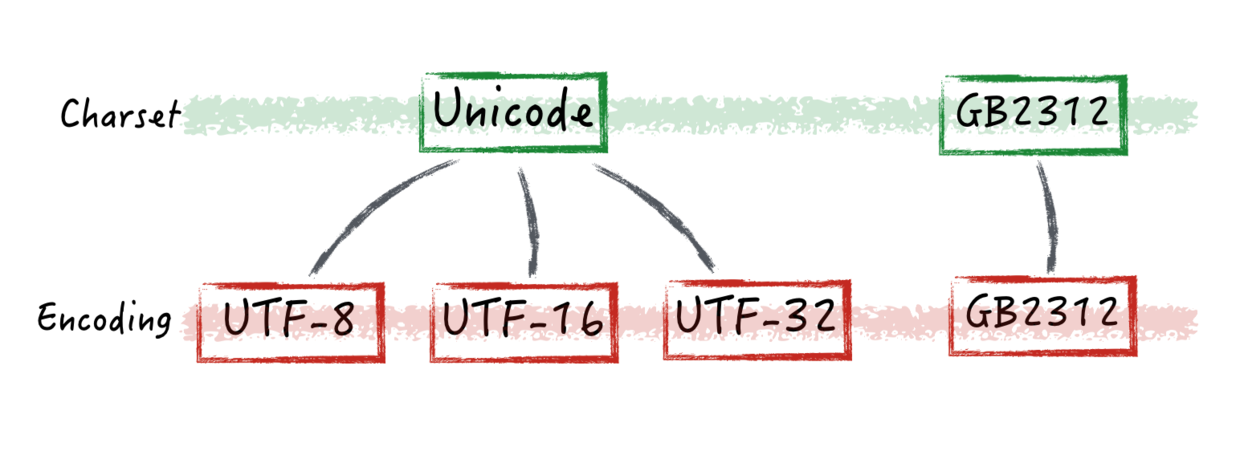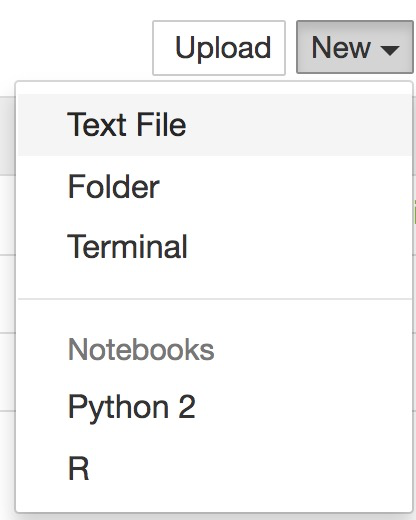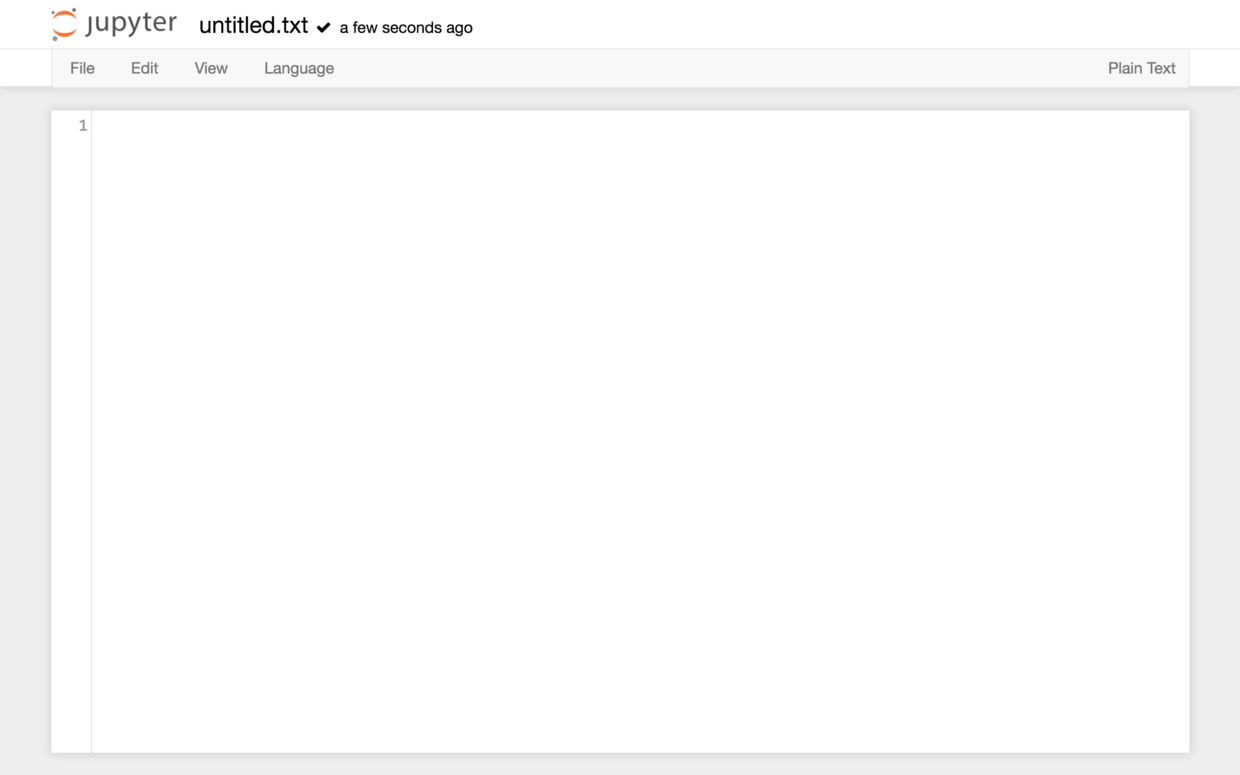### 5.4.4 执行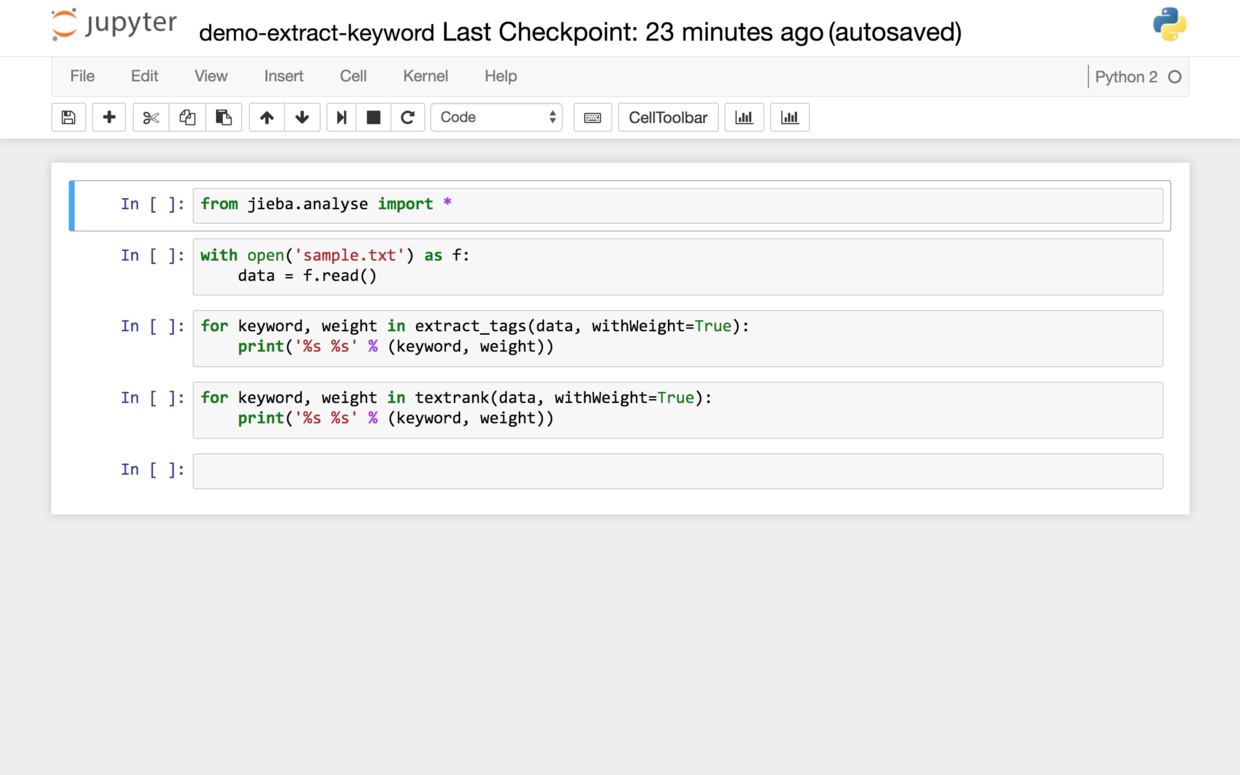``from jieba.analyse import *``

``````with open('sample.txt') as f:

``````for keyword, weight in extract_tags(data, withWeight=True):
print('%s %s' % (keyword, weight))``````

``````Building prefix dict from the default dictionary ...
Prefix dict has been built succesfully.``````

``````优步 0.280875594782

10 0.0526641740216

``````for keyword, weight in extract_tags(data, topK=10, withWeight=True):
print('%s %s' % (keyword, weight))``````

``````for keyword, weight in textrank(data, withWeight=True):
print('%s %s' % (keyword, weight))``````

``````优步 1.0

### 5.4.5 原理

A可能就是虚词，或者全部文档共享的主题词。而B只在3篇文档中出现，因此很有可能是个关键词。

TF-idf讲完了，下面我们说说TextRank。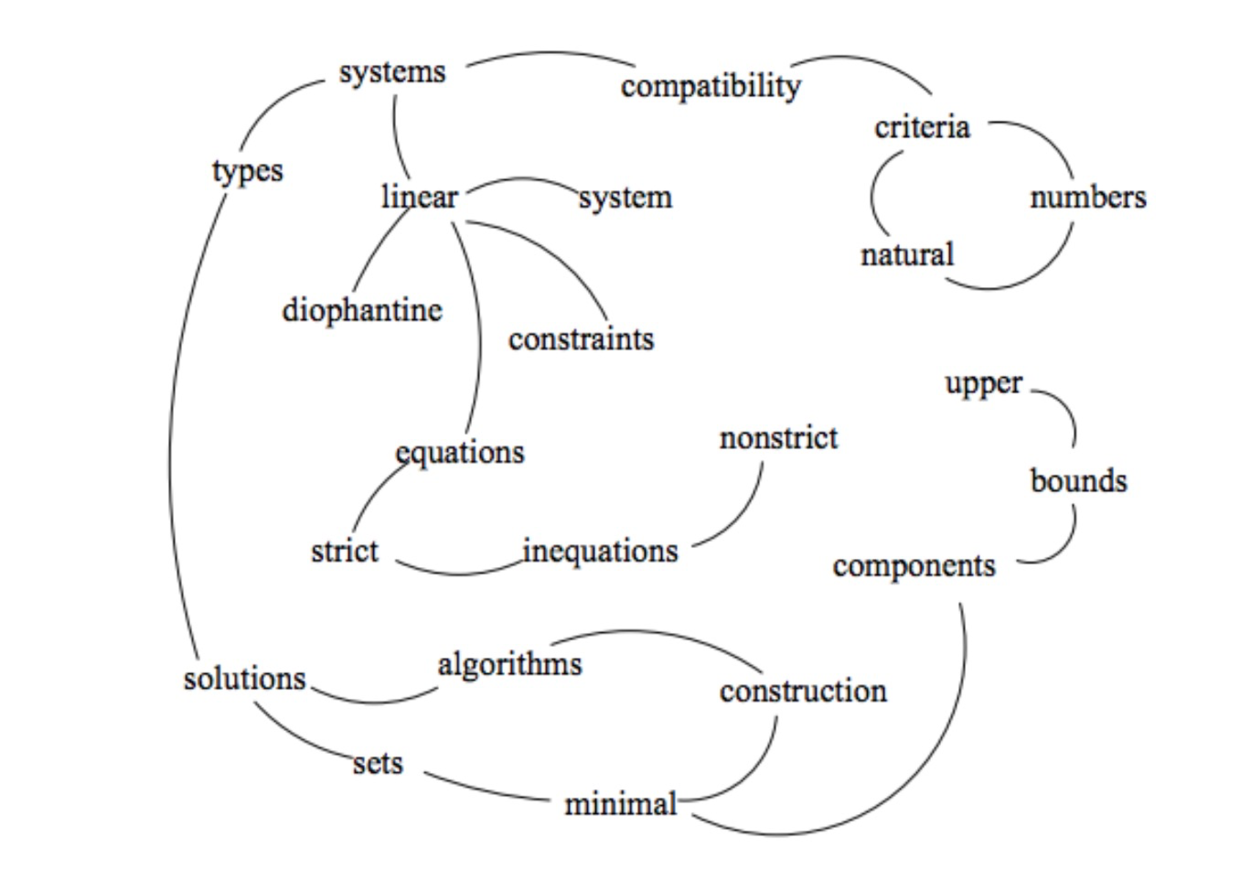TextRank首先会提取词汇，形成节点；然后依据词汇的关联，建立链接。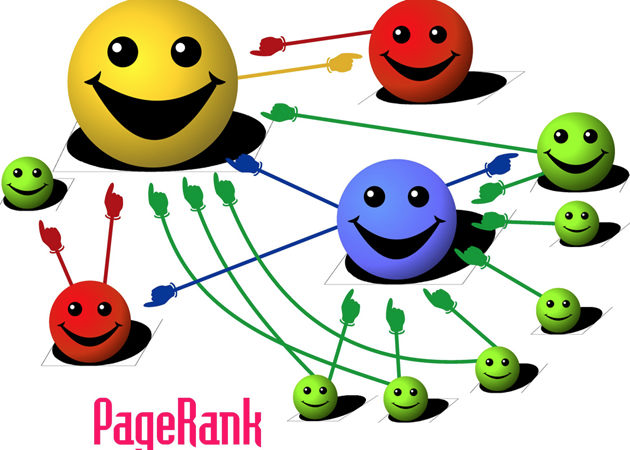## 5.5 如何用Python处理自然语言？（Spacy与Word Embedding）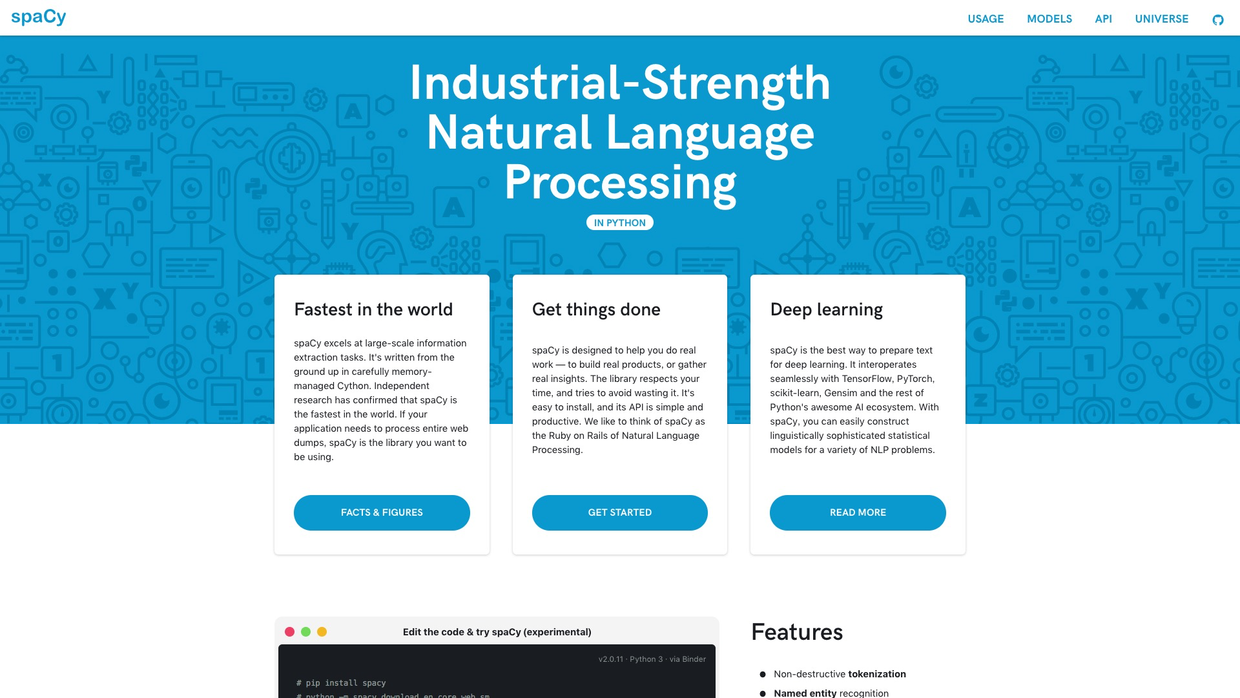### 5.5.1 盲维

To the one with a hammer, everything looks like a nail. （手中有锤，看什么都像钉）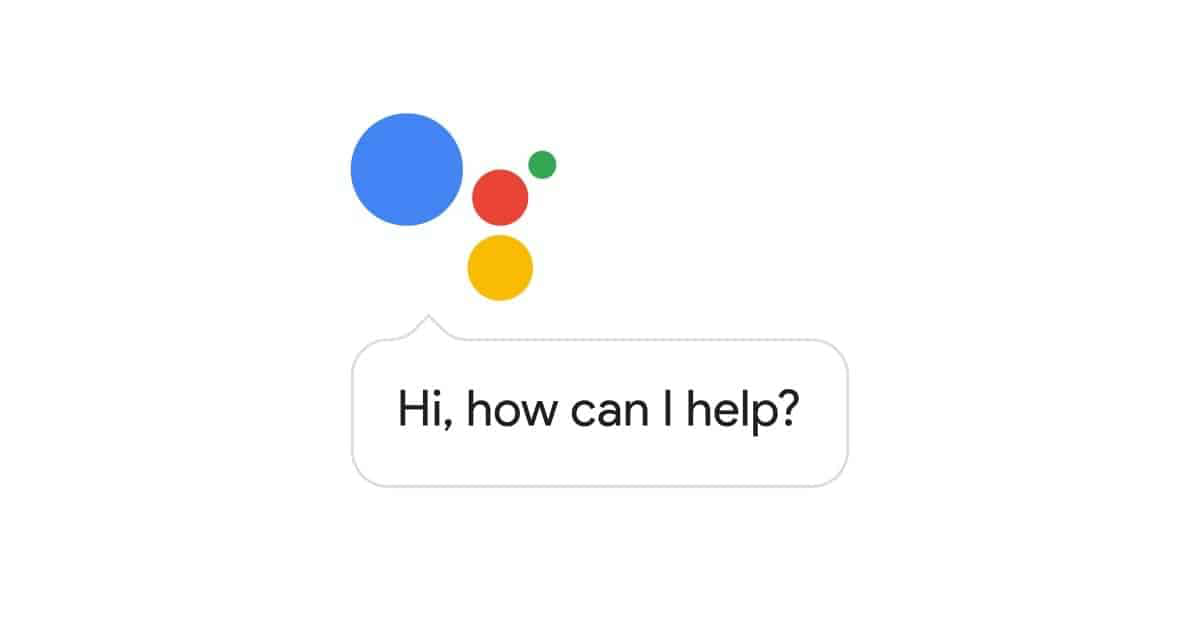### 5.5.2 工具

Spacy 的 Slogan，是这样的：

Industrial-Strength Natural Language Processing. （工业级别的自然语言处理）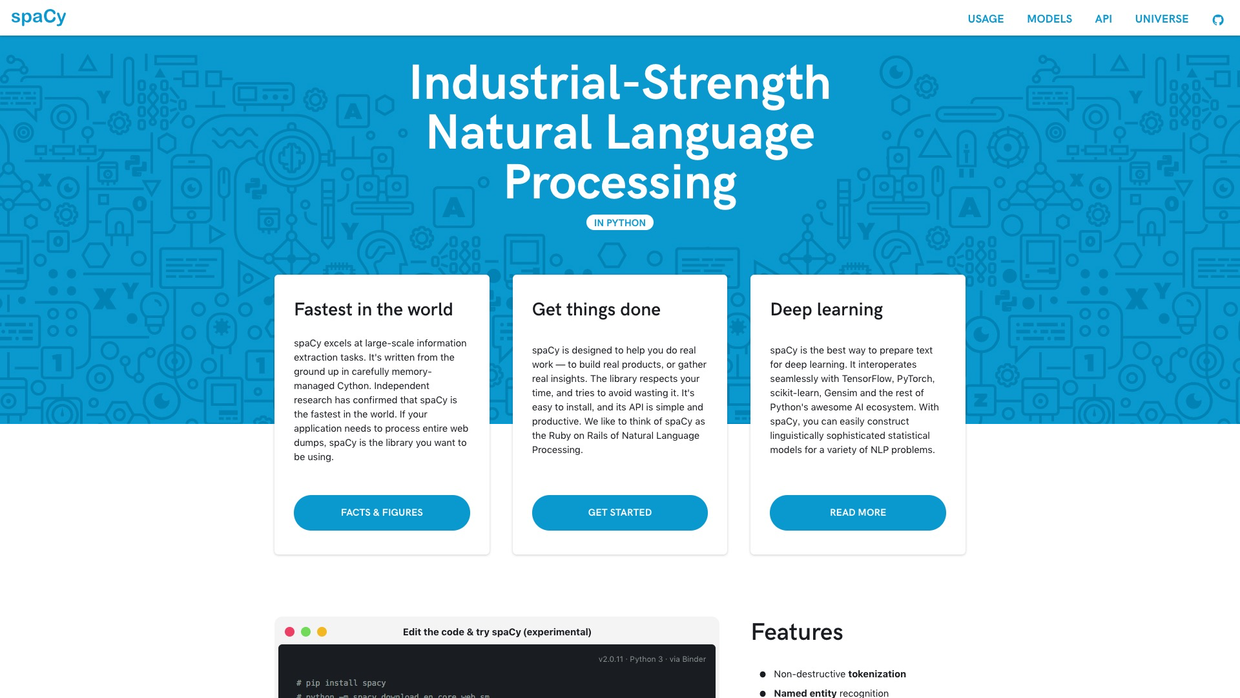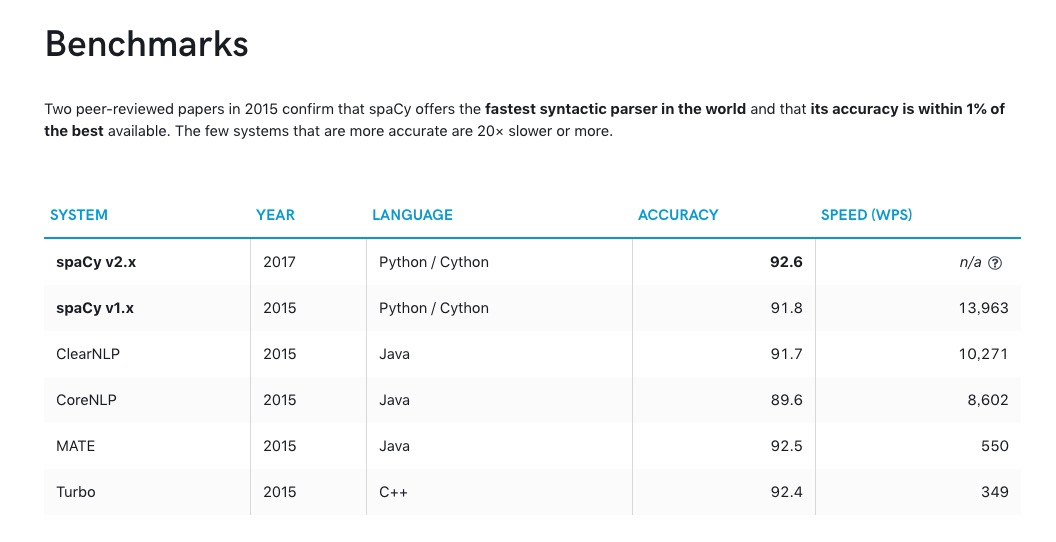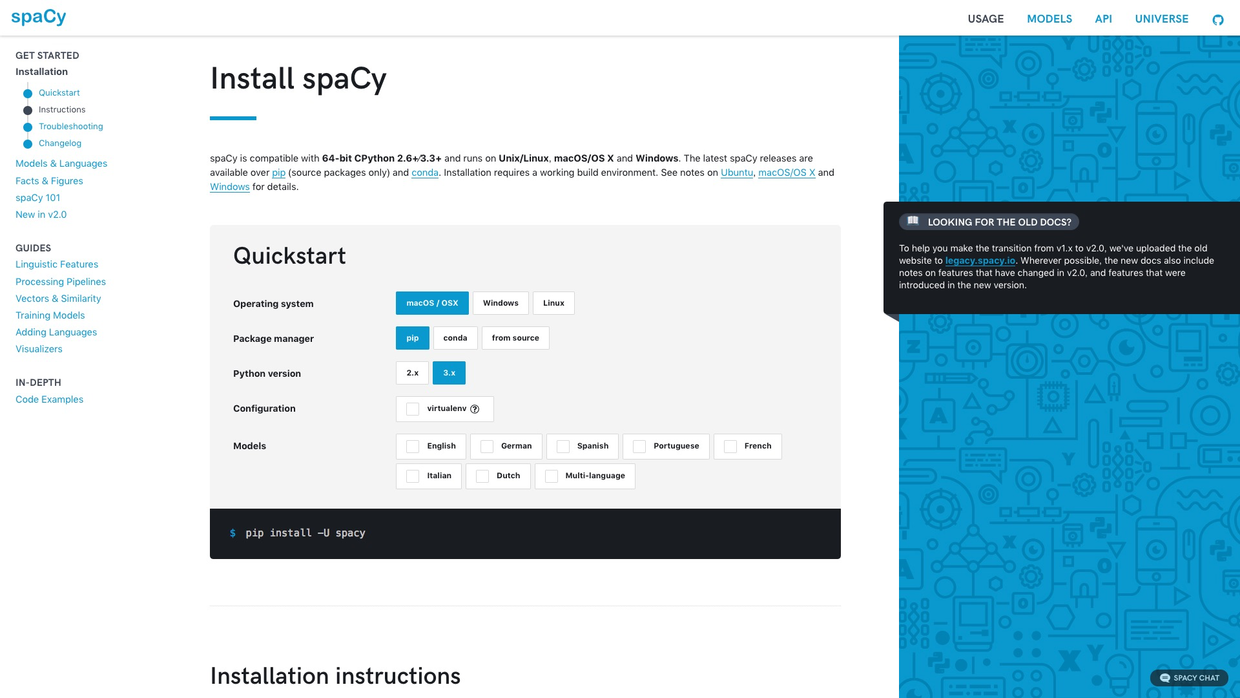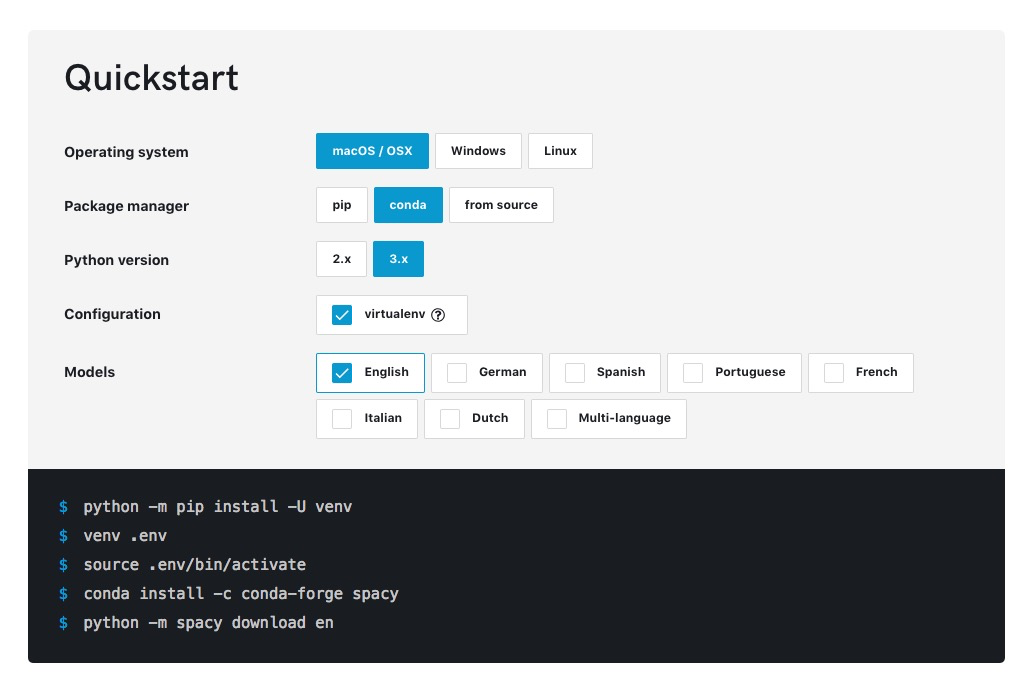Spacy的功能有很多。

• 词性分析
• 命名实体识别
• 依赖关系刻画
• 词嵌入向量的近似度计算
• 词语降维和可视化

### 5.5.3 环境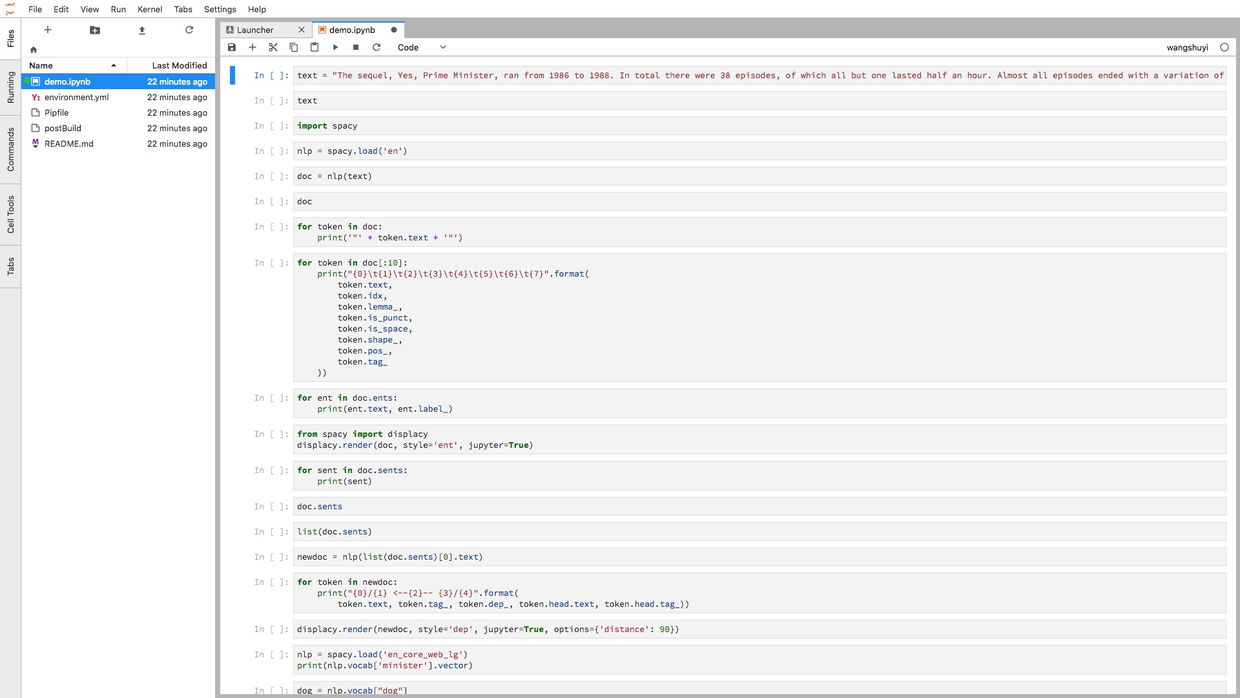• 代码单元直接鼠标拖动；
• 一个浏览器标签，可打开多个Notebook，而且分别使用不同的Kernel；
• 提供实时渲染的Markdown编辑器；
• 完整的文件浏览器；
• CSV数据文件快速浏览
• ……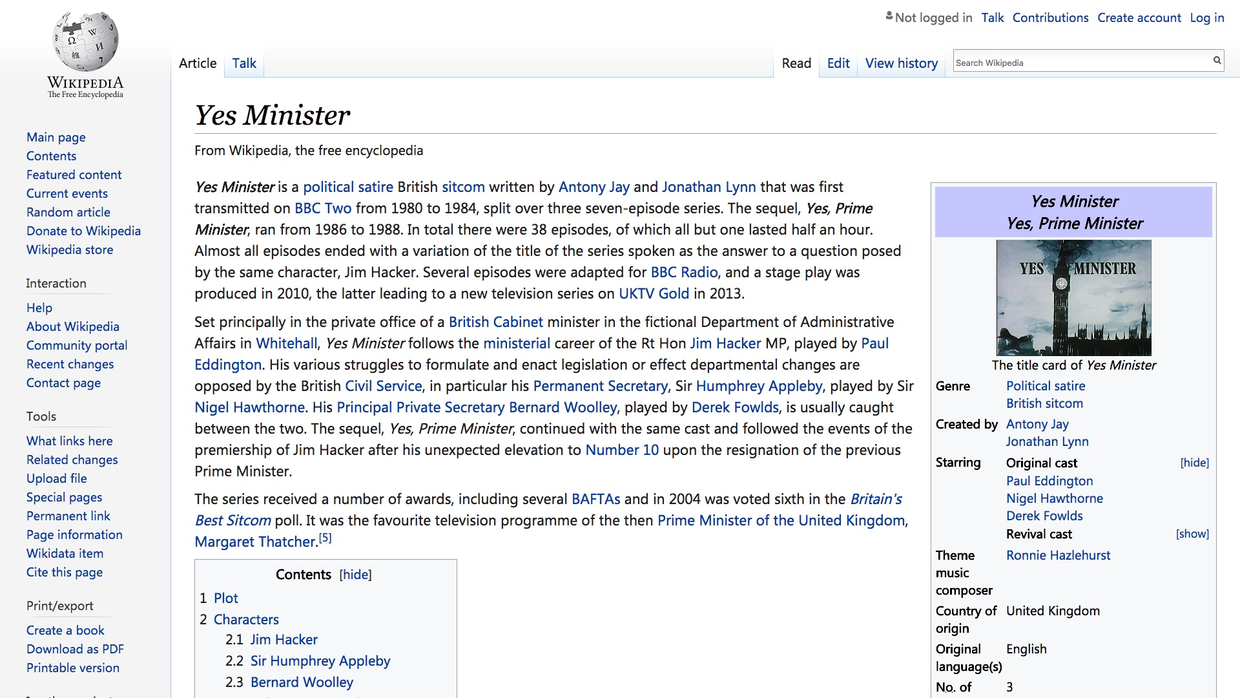### 5.5.4 实践

``text = "The sequel, Yes, Prime Minister, ran from 1986 to 1988. In total there were 38 episodes, of which all but one lasted half an hour. Almost all episodes ended with a variation of the title of the series spoken as the answer to a question posed by the same character, Jim Hacker. Several episodes were adapted for BBC Radio, and a stage play was produced in 2010, the latter leading to a new television series on UKTV Gold in 2013."``

``text``
``'The sequel, Yes, Prime Minister, ran from 1986 to 1988. In total there were 38 episodes, of which all but one lasted half an hour. Almost all episodes ended with a variation of the title of the series spoken as the answer to a question posed by the same character, Jim Hacker. Several episodes were adapted for BBC Radio, and a stage play was produced in 2010, the latter leading to a new television series on UKTV Gold in 2013.'``

``import spacy``

``nlp = spacy.load('en')``

``doc = nlp(text)``

``doc``
``The sequel, Yes, Prime Minister, ran from 1986 to 1988. In total there were 38 episodes, of which all but one lasted half an hour. Almost all episodes ended with a variation of the title of the series spoken as the answer to a question posed by the same character, Jim Hacker. Several episodes were adapted for BBC Radio, and a stage play was produced in 2010, the latter leading to a new television series on UKTV Gold in 2013.``

``````for token in doc:
print('"' + token.text + '"')``````

``````"The"
"sequel"
","
"Yes"
","
"Prime"
"Minister"
","
"ran"
"from"
"1986"
"to"
"1988"
"."
"In"
"total"
"there"
"were"
"38"
"episodes"
","
"of"
"which"
"all"
"but"
"one"
"lasted"
"half"
"an"
"hour"
"."
"Almost"
"all"
"episodes"
"ended"
"with"
"a"
"variation"
"of"
"the"
"title"
"of"
"the"
"series"
"spoken"
"as"
"the"
"to"
"a"
"question"
"posed"
"by"
"the"
"same"
"character"
","
"Jim"
"Hacker"
"."
"Several"
"episodes"
"were"
"for"
"BBC"
","
"and"
"a"
"stage"
"play"
"was"
"produced"
"in"
"2010"
","
"the"
"latter"
"to"
"a"
"new"
"television"
"series"
"on"
"UKTV"
"Gold"
"in"
"2013"
"."``````

• 文本
• 索引值（即在原文中的定位）
• 词元(lemma)
• 是否为标点符号
• 是否为空格
• 词性
• 标记
``````for token in doc[:10]:
print("{0}\t{1}\t{2}\t{3}\t{4}\t{5}\t{6}\t{7}".format(
token.text,
token.idx,
token.lemma_,
token.is_punct,
token.is_space,
token.shape_,
token.pos_,
token.tag_
))``````

``````The 0   the False   False   Xxx DET DT
sequel  4   sequel  False   False   xxxx    NOUN    NN
,   10  ,   True    False   ,   PUNCT   ,
Yes 12  yes False   False   Xxx INTJ    UH
,   15  ,   True    False   ,   PUNCT   ,
Prime   17  prime   False   False   Xxxxx   PROPN   NNP
Minister    23  minister    False   False   Xxxxx   PROPN   NNP
,   31  ,   True    False   ,   PUNCT   ,
ran 33  run False   False   xxx VERB    VBD
from    37  from    False   False   xxxx    ADP IN``````

``````for ent in doc.ents:
print(ent.text, ent.label_)``````
``````1986 to 1988 DATE
38 CARDINAL
one CARDINAL
half an hour TIME
Jim Hacker PERSON
2010 DATE
UKTV Gold ORG
2013 DATE``````

``````from spacy import displacy
displacy.render(doc, style='ent', jupyter=True)``````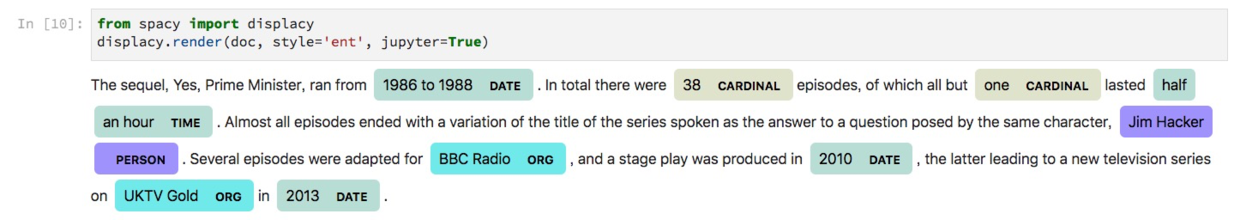``````for sent in doc.sents:
print(sent)``````
``````The sequel, Yes, Prime Minister, ran from 1986 to 1988.
In total there were 38 episodes, of which all but one lasted half an hour.
Almost all episodes ended with a variation of the title of the series spoken as the answer to a question posed by the same character, Jim Hacker.
Several episodes were adapted for BBC Radio, and a stage play was produced in 2010, the latter leading to a new television series on UKTV Gold in 2013.``````

``doc.sents``
``<generator at 0x116e95e18>``

``list(doc.sents)``
``````[The sequel, Yes, Prime Minister, ran from 1986 to 1988.,
In total there were 38 episodes, of which all but one lasted half an hour.,
Almost all episodes ended with a variation of the title of the series spoken as the answer to a question posed by the same character, Jim Hacker.,
Several episodes were adapted for BBC Radio, and a stage play was produced in 2010, the latter leading to a new television series on UKTV Gold in 2013.]``````

``newdoc = nlp(list(doc.sents).text)``

``````for token in newdoc:
print("{0}/{1} <--{2}-- {3}/{4}".format(
``````The/DT <--det-- sequel/NN
sequel/NN <--nsubj-- ran/VBD
,/, <--punct-- sequel/NN
Yes/UH <--intj-- sequel/NN
,/, <--punct-- sequel/NN
Prime/NNP <--compound-- Minister/NNP
Minister/NNP <--appos-- sequel/NN
,/, <--punct-- sequel/NN
ran/VBD <--ROOT-- ran/VBD
from/IN <--prep-- ran/VBD
1986/CD <--pobj-- from/IN
to/IN <--prep-- from/IN
1988/CD <--pobj-- to/IN
./. <--punct-- ran/VBD``````

``displacy.render(newdoc, style='dep', jupyter=True, options={'distance': 90})``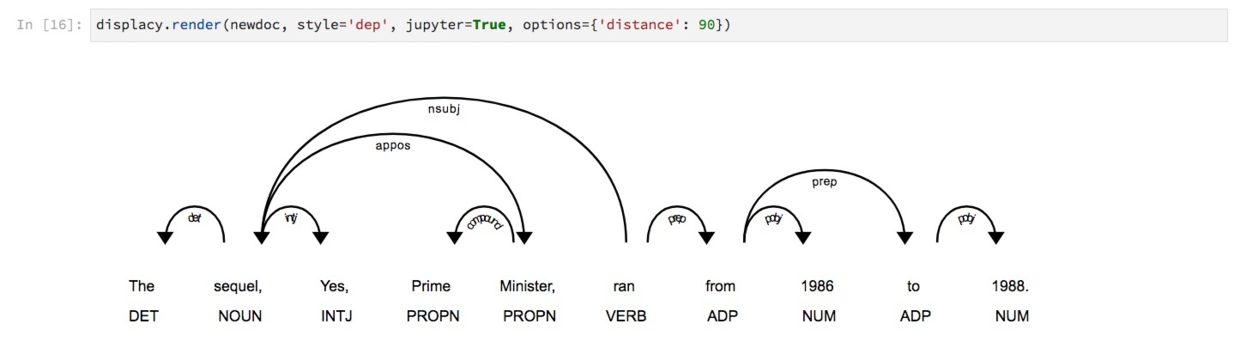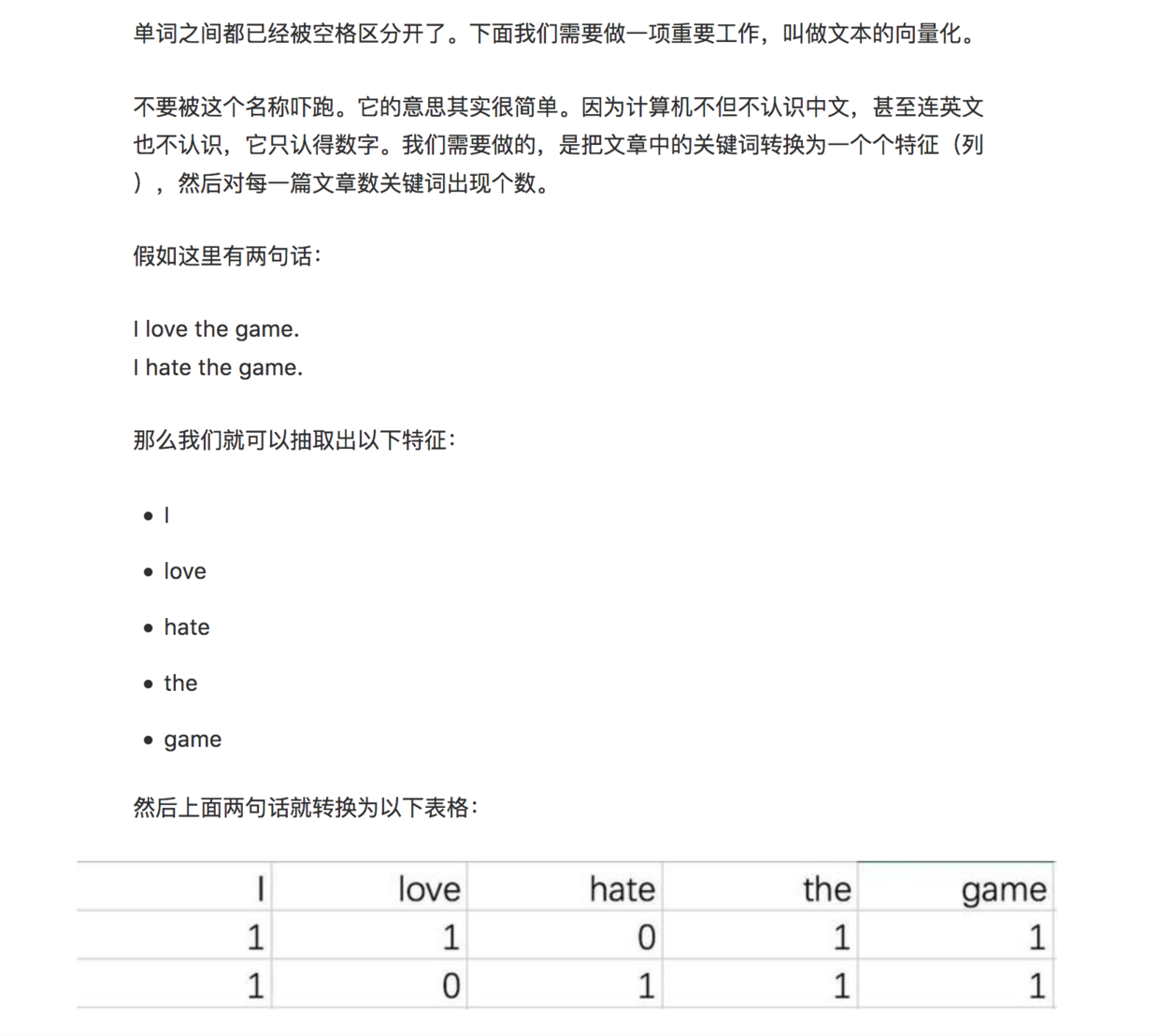``? - woman = king - queen``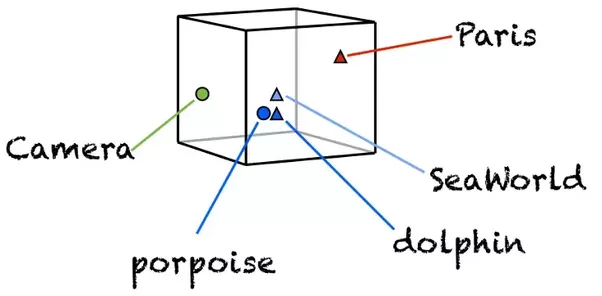``nlp = spacy.load('en_core_web_lg')``

``print(nlp.vocab['minister'].vector)``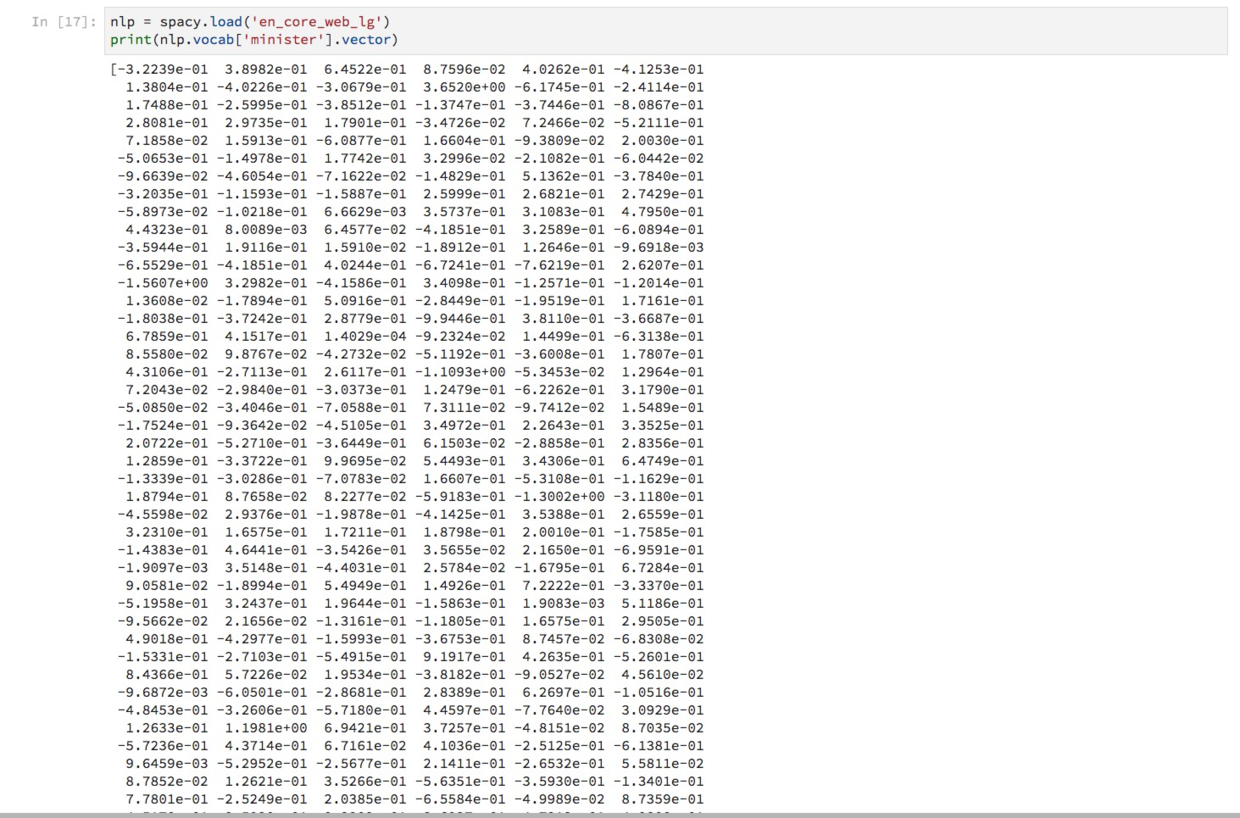``````dog = nlp.vocab["dog"]
cat = nlp.vocab["cat"]
apple = nlp.vocab["apple"]
orange = nlp.vocab["orange"]``````

``dog.similarity(cat)``
``0.80168545``

``dog.similarity(apple)``
``0.26339027``

“狗”和“橘子”呢？

``dog.similarity(orange)``
``0.2742508``

``apple.similarity(orange)``
``0.5618917``

``from scipy.spatial.distance import cosine``

``1 - cosine(dog.vector, cat.vector)``
``0.8016855120658875``

``````def vector_similarity(x, y):
return 1 - cosine(x, y)``````

``vector_similarity(dog.vector, apple.vector)``
``0.2633902430534363``

``? - woman = king - queen``

``guess_word = king - queen + woman``

``````def make_guess_word(words):
[first, second, third] = words
return nlp.vocab[first].vector - nlp.vocab[second].vector + nlp.vocab[third].vector``````

``````def get_similar_word(words, scope=nlp.vocab):

guess_word = make_guess_word(words)

similarities = []

for word in scope:
if not word.has_vector:
continue

similarity = vector_similarity(guess_word, word.vector)
similarities.append((word, similarity))

similarities = sorted(similarities, key=lambda item: -item)
print([word.text for word in similarities[:10]])``````

``? - woman = king - queen``

``guess_word = king - queen + woman``

``words = ["king", "queen", "woman"]``

``get_similar_word(words)``

``['MAN', 'Man', 'mAn', 'MAn', 'MaN', 'man', 'mAN', 'WOMAN', 'womAn', 'WOman']``

``? - England = Paris - London``

``guess_word = Paris - London + England``

``words = ["Paris", "London", "England"]``

``get_similar_word(words)``
``['france', 'FRANCE', 'France', 'Paris', 'paris', 'PARIS', 'EUROPE', 'EUrope', 'europe', 'Europe']``

``import numpy as np``

``embedding = np.array([])``

``word_list = []``

• 如果是标点，丢弃；
• 如果词汇已经在词语列表中，丢弃。
``````for token in doc:
if not(token.is_punct) and not(token.text in word_list):
word_list.append(token.text)``````

``word_list``
``````['The',
'sequel',
'Yes',
'Prime',
'Minister',
'ran',
'from',
'1986',
'to',
'1988',
'In',
'total',
'there',
'were',
'38',
'episodes',
'of',
'which',
'all',
'but',
'one',
'lasted',
'half',
'an',
'hour',
'Almost',
'ended',
'with',
'a',
'variation',
'the',
'title',
'series',
'spoken',
'as',
'question',
'posed',
'by',
'same',
'character',
'Jim',
'Hacker',
'Several',
'for',
'BBC',
'and',
'stage',
'play',
'was',
'produced',
'in',
'2010',
'latter',
'new',
'television',
'on',
'UKTV',
'Gold',
'2013']``````

``````for word in word_list:
embedding = np.append(embedding, nlp.vocab[word].vector)``````

``embedding.shape``
``(18900,)``

``embedding = embedding.reshape(len(word_list), -1)``

``embedding.shape``
``(63, 300)``

63个词汇，每个长度300，这就对了。

``from sklearn.manifold import TSNE``

``tsne = TSNE()``

tsne的作用，是把高维度的词向量（300维）压缩到二维平面上。我们执行这个转换过程：

``low_dim_embedding = tsne.fit_transform(embedding)``

``````import matplotlib.pyplot as plt
%pylab inline``````

``````def plot_with_labels(low_dim_embs, labels, filename='tsne.pdf'):
assert low_dim_embs.shape >= len(labels), "More labels than embeddings"
plt.figure(figsize=(18, 18))  # in inches
for i, label in enumerate(labels):
x, y = low_dim_embs[i, :]
plt.scatter(x, y)
plt.annotate(label,
xy=(x, y),
xytext=(5, 2),
textcoords='offset points',
ha='right',
va='bottom')
plt.savefig(filename)``````

``plot_with_labels(low_dim_embedding, word_list)``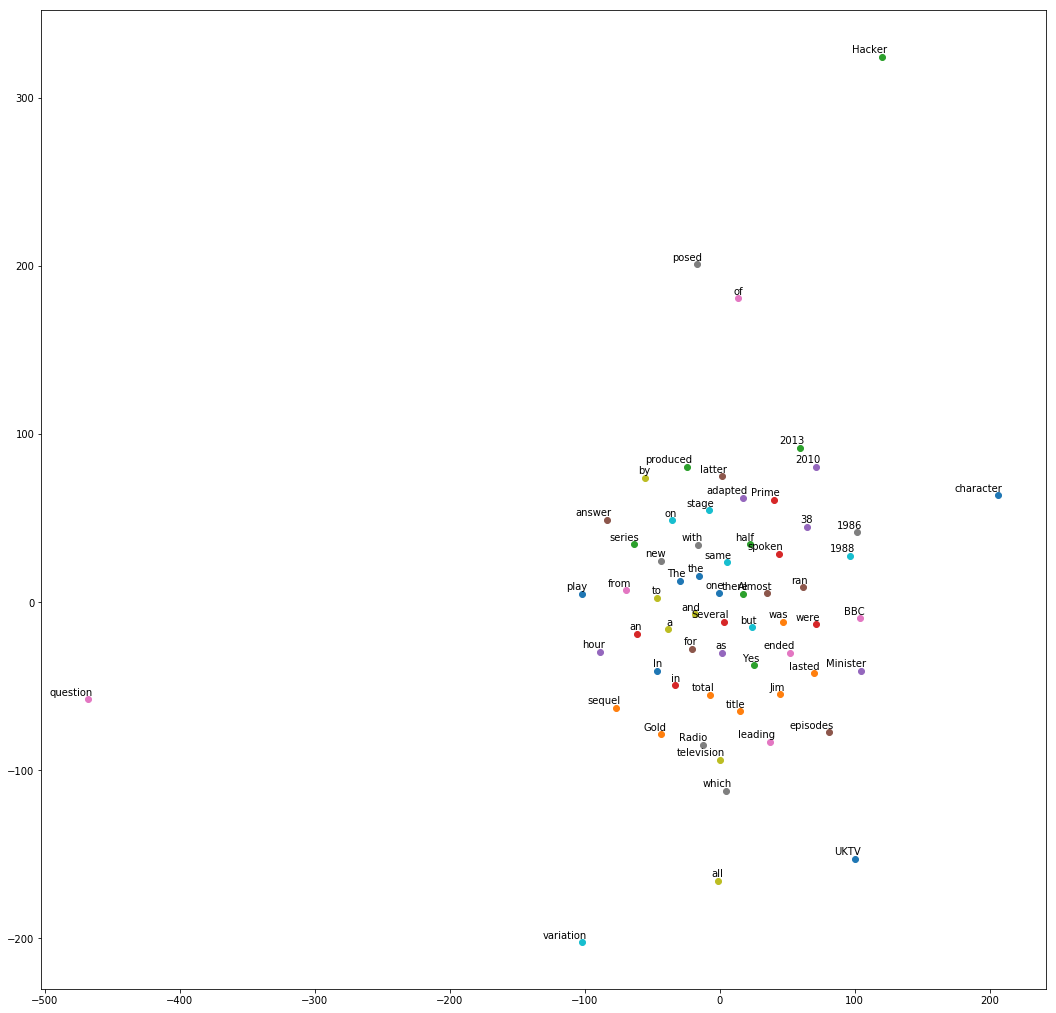• 年份
• 同一单词的大小写形式
• a 和 an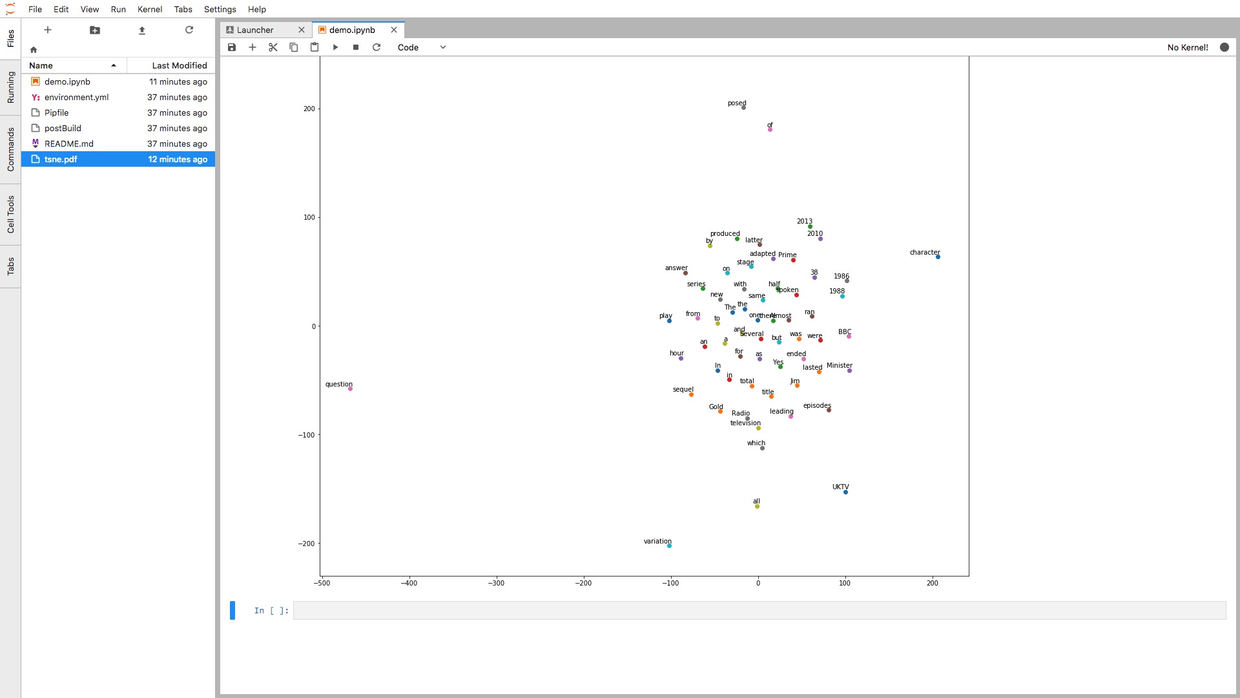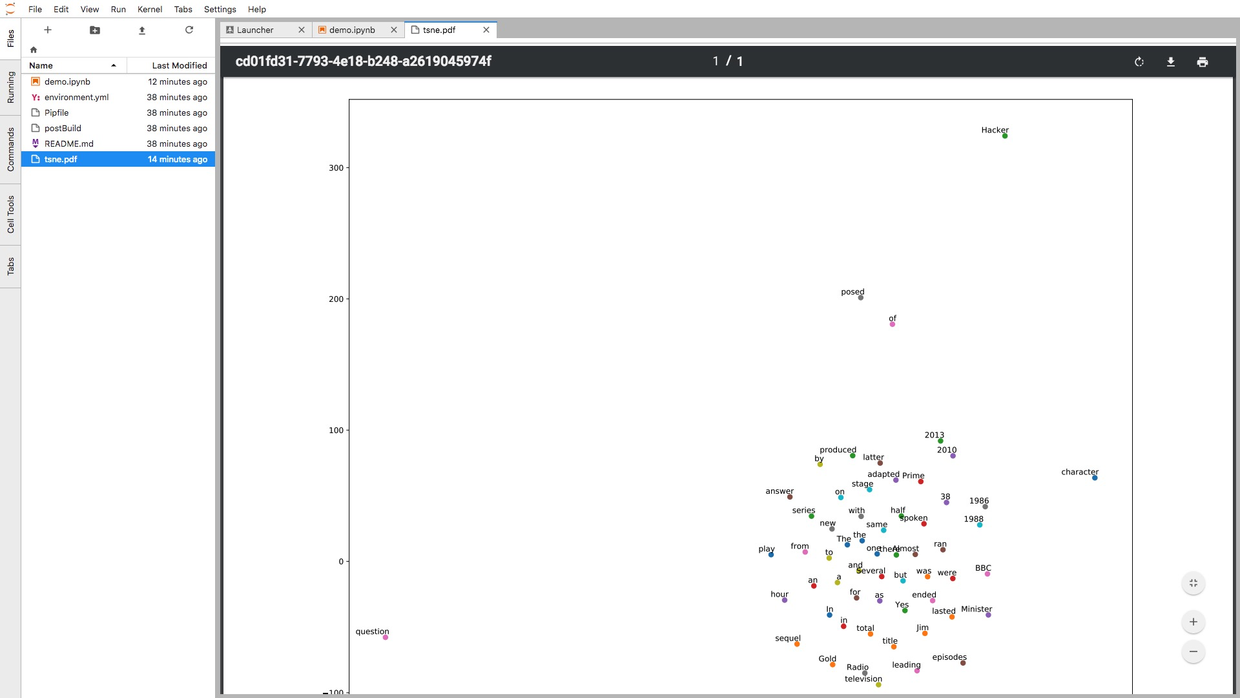### 5.5.5 源码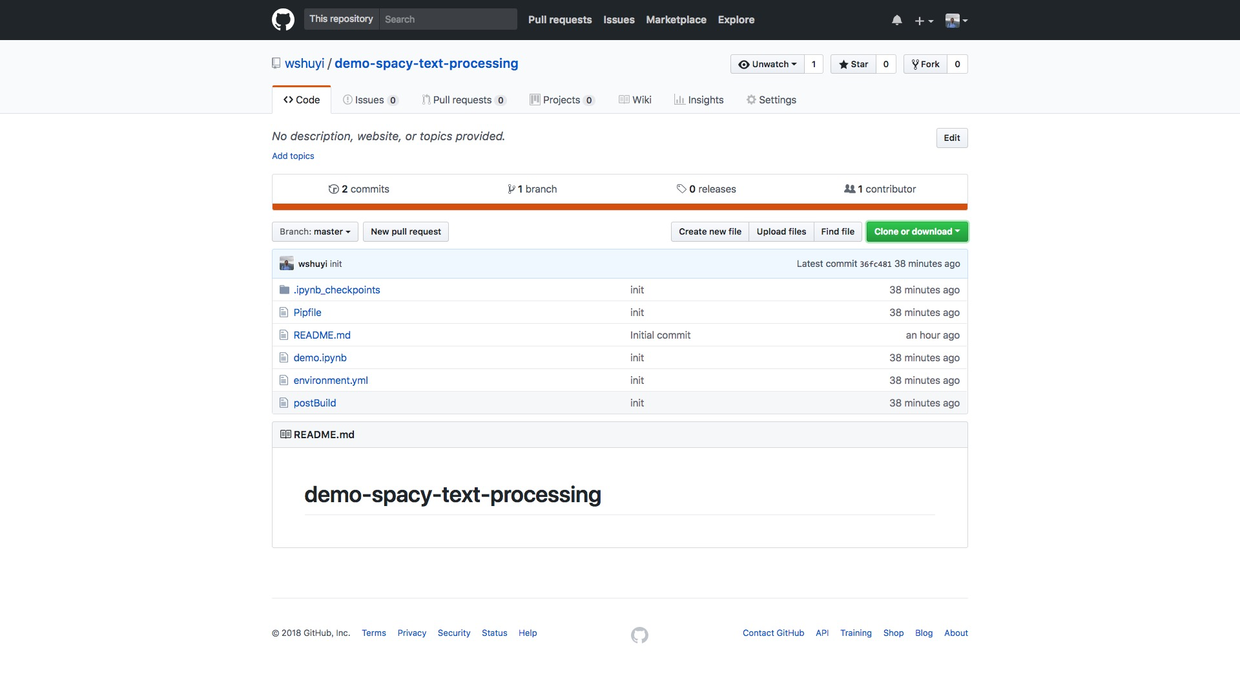• 词性分析
• 命名实体识别
• 依赖关系刻画
• 词嵌入向量的近似度计算
• 词语降维和可视化

## 5.6 如何用 Python 和 gensim 调用中文词嵌入预训练模型？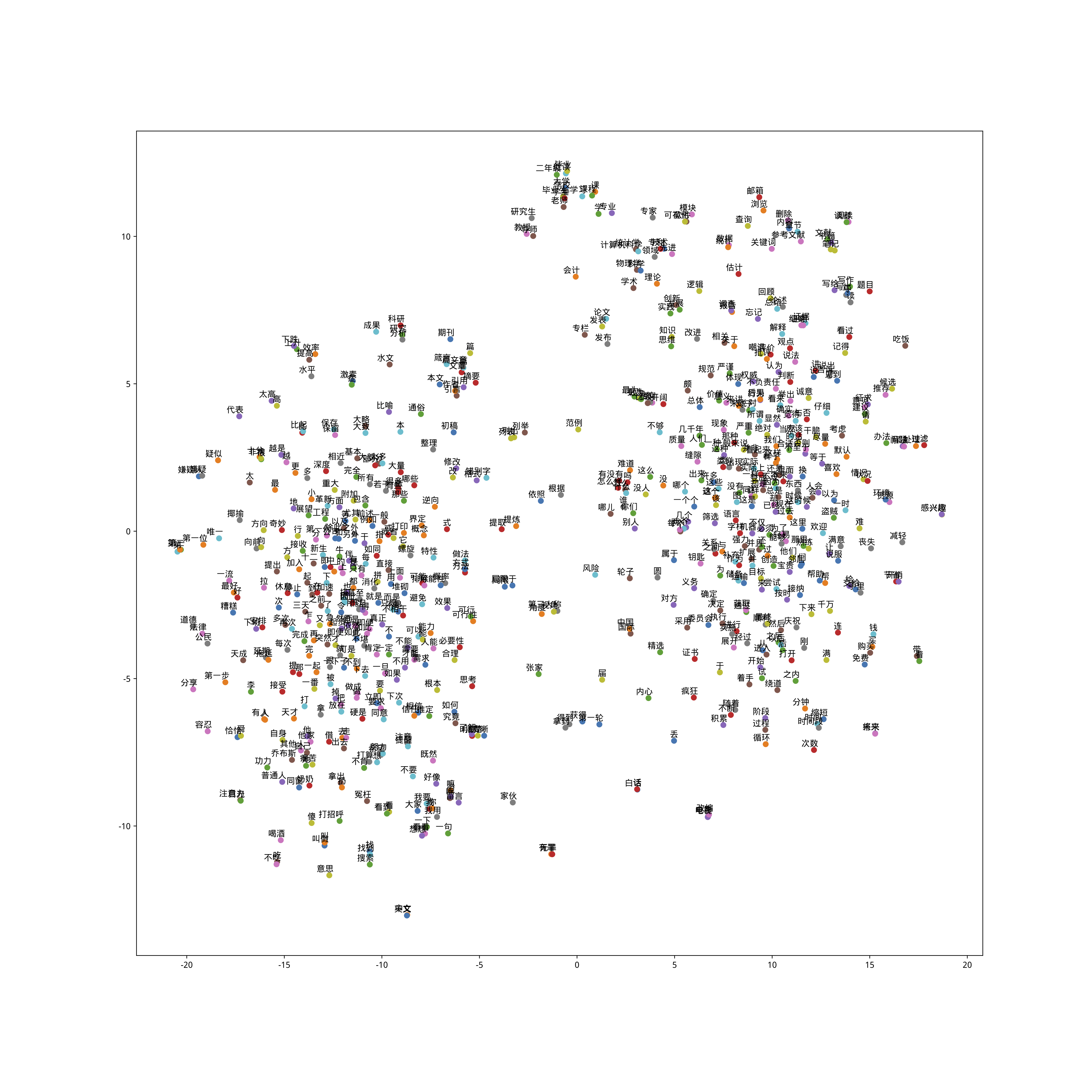### 5.6.1 疑问

``dog.similarity(cat)``
``0.80168545``

``dog.similarity(orange)``
``0.2742508``

``? - woman = king - queen``

``guess_word = king - queen + woman``

``words = ["king", "queen", "woman"]``

``['MAN', 'Man', 'mAn', 'MAn', 'MaN', 'man', 'mAN', 'WOMAN', 'womAn', 'WOman']``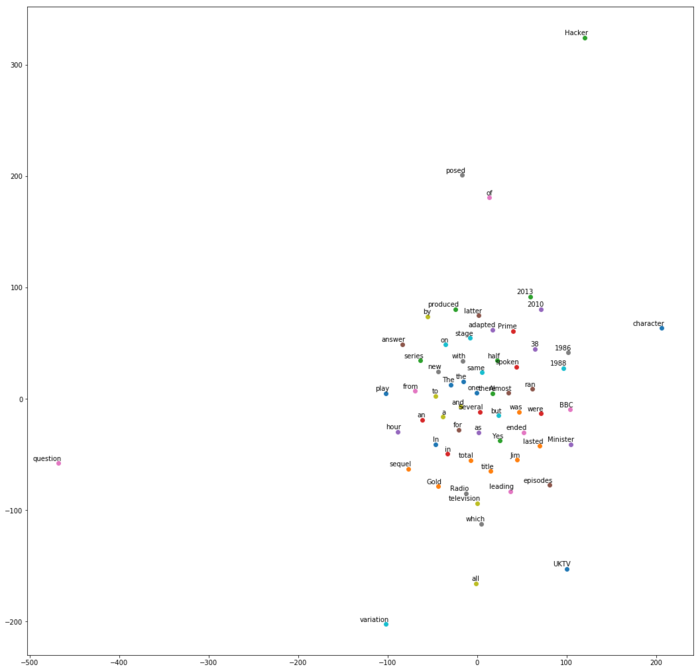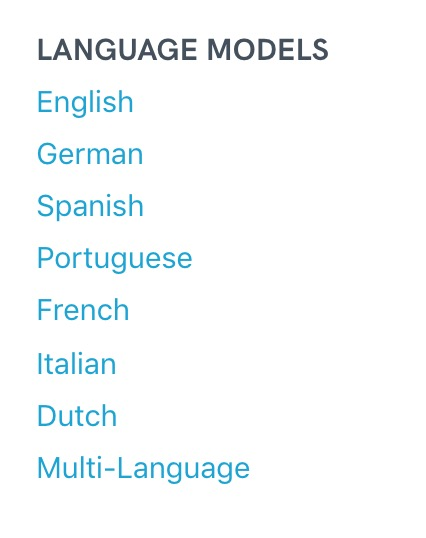### 5.6.2 工具

Topic modelling for humans.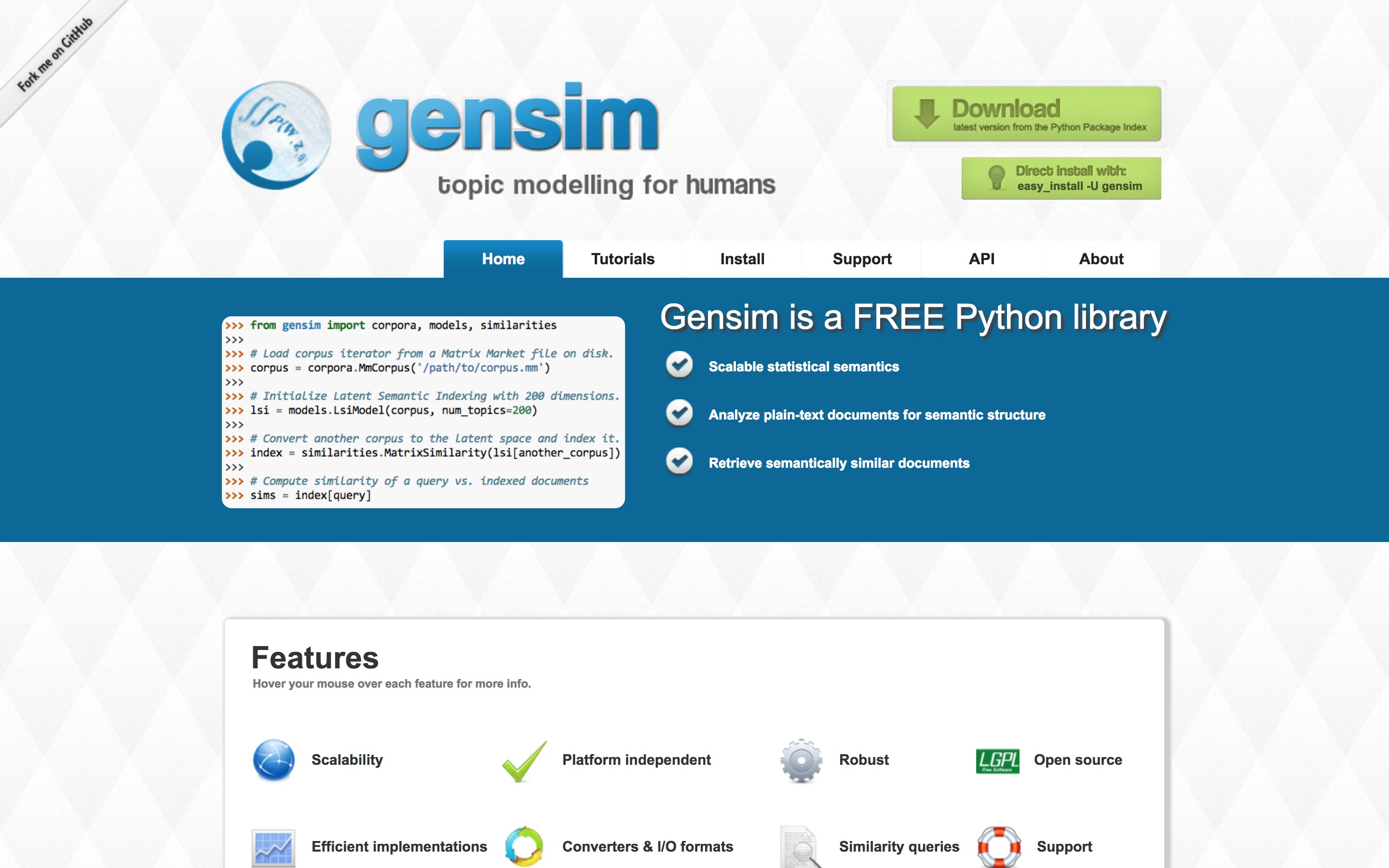Gensim 包很强大，甚至可以直接用来做情感分析主题挖掘（关于主题挖掘的含义，可以参考我的《如何用Python从海量文本抽取主题？》（6.2）一文）。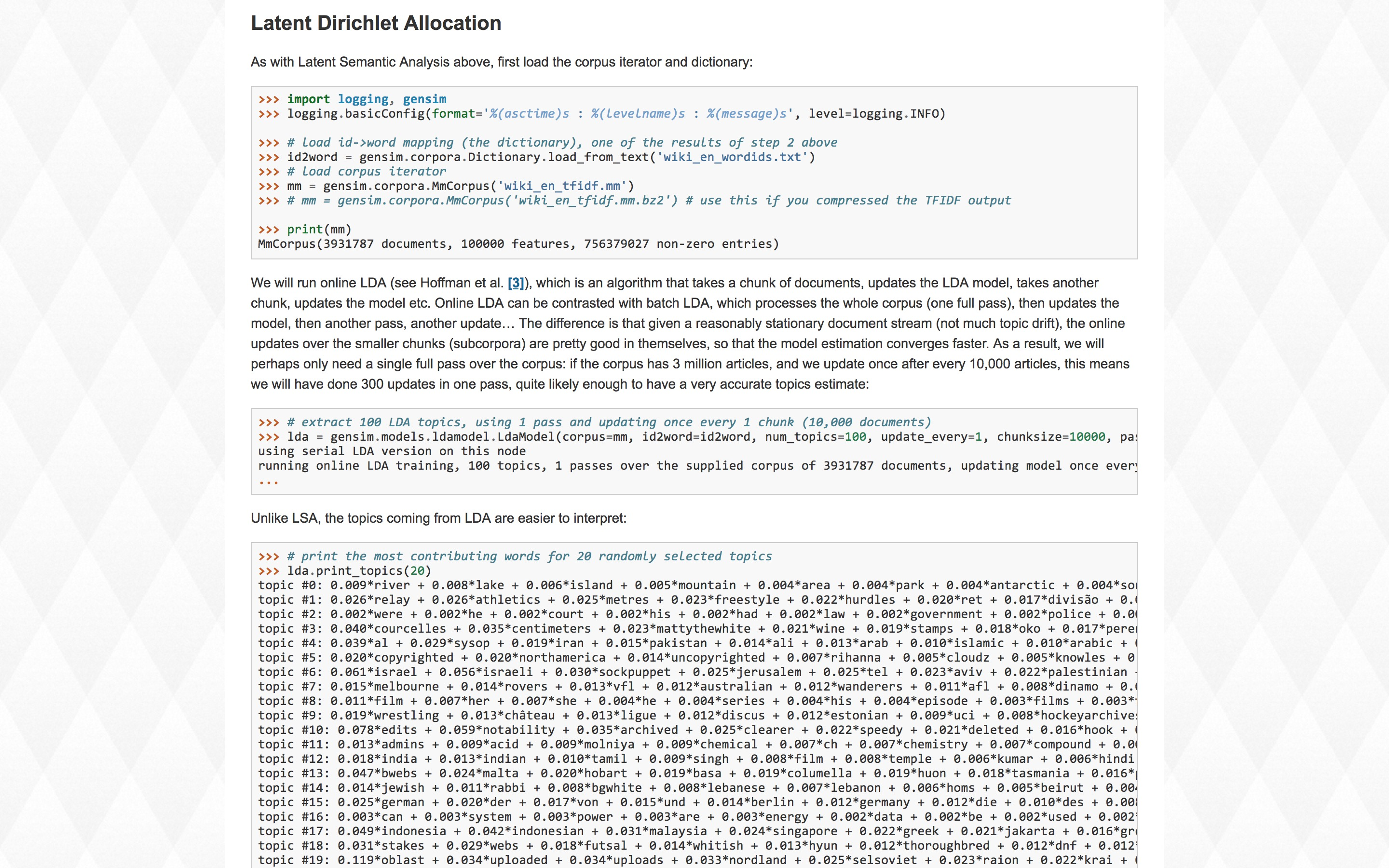### 5.6.3 视频教程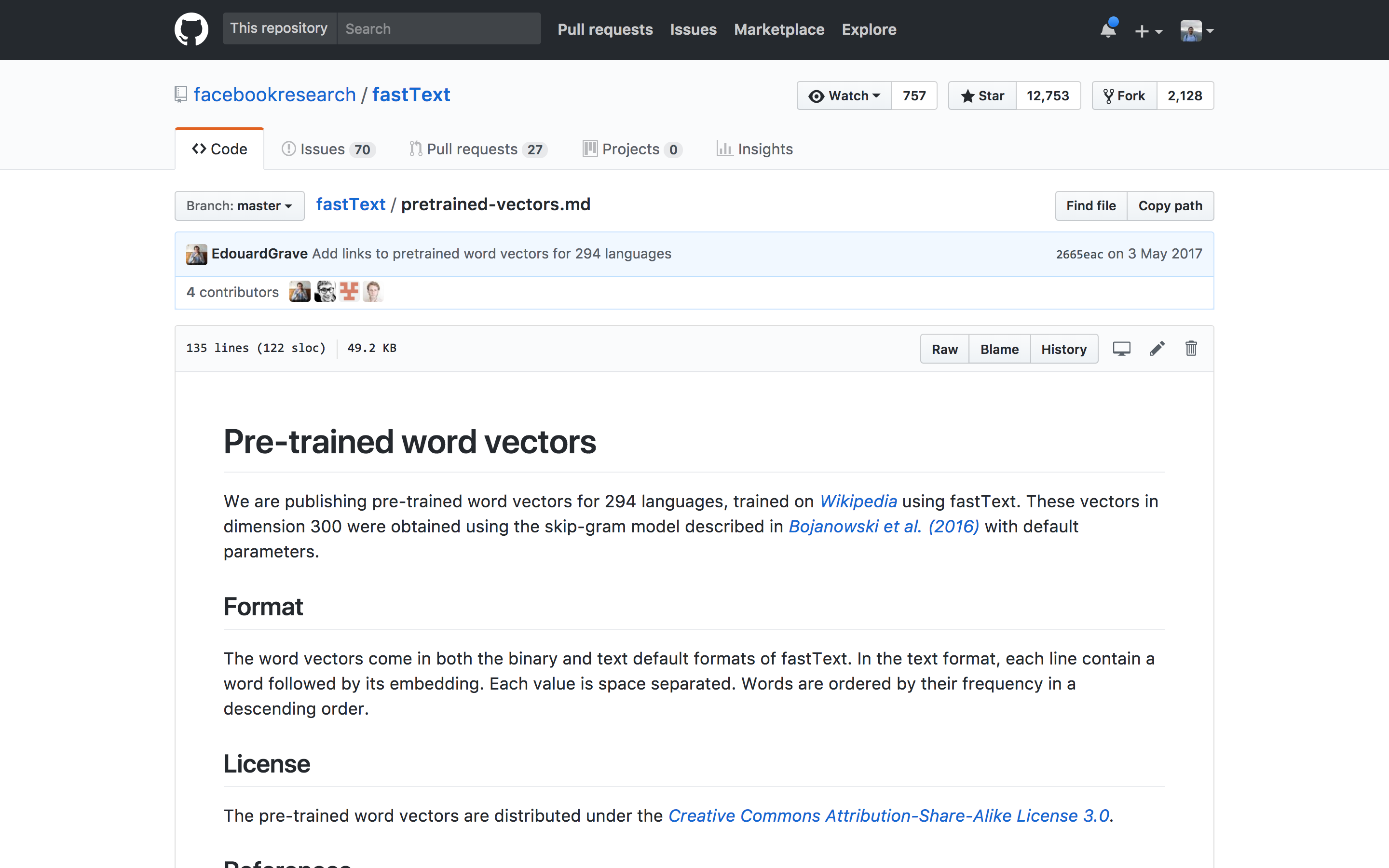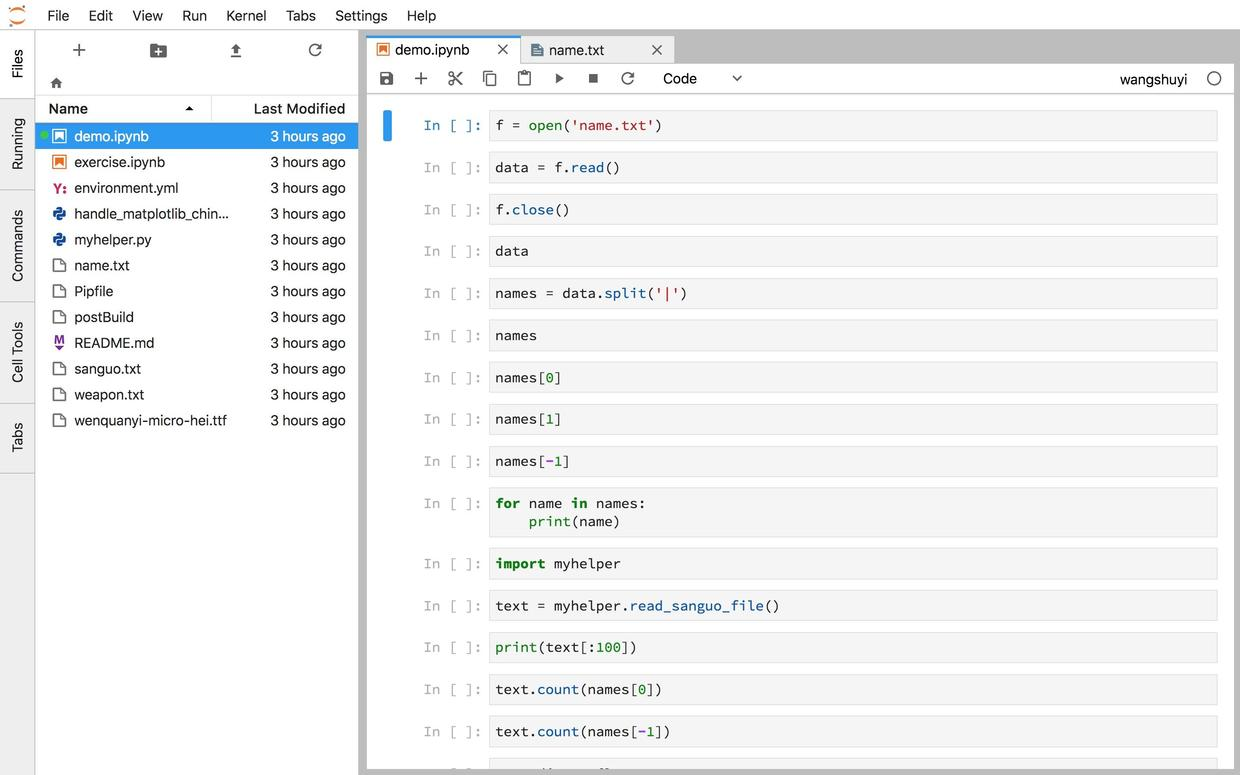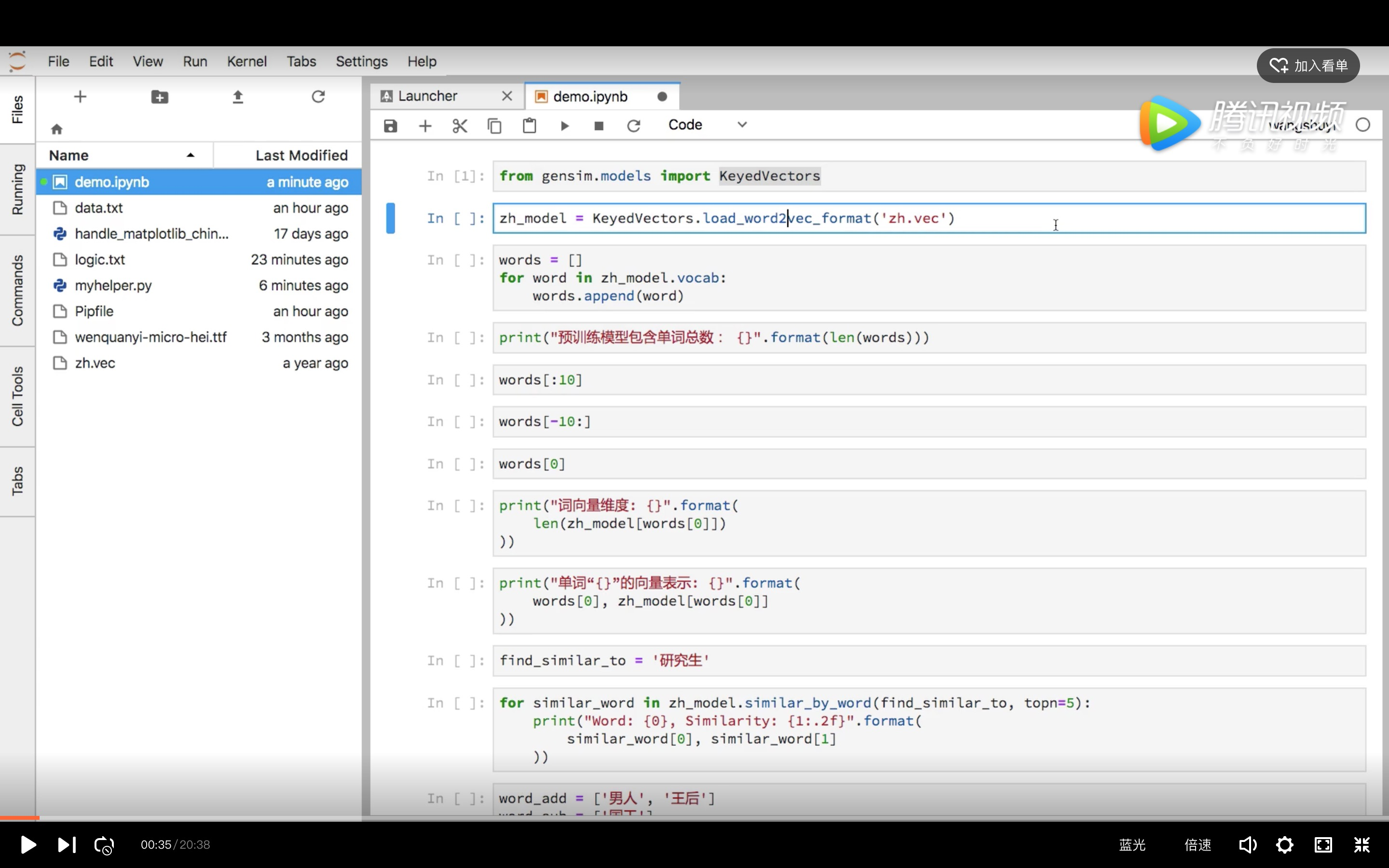• 如何用 gensim 建立语言模型；
• 如何把词嵌入预训练模型读入；
• 如何根据语义，查找某单词近似词汇列表；
• 如何利用语义计算，进行查询；
• 如何用字符串替换与结巴分词对中文文本做预处理；
• 如何用 tsne 将高维词向量压缩到低维；
• 如何可视化压缩到低维的词汇集合；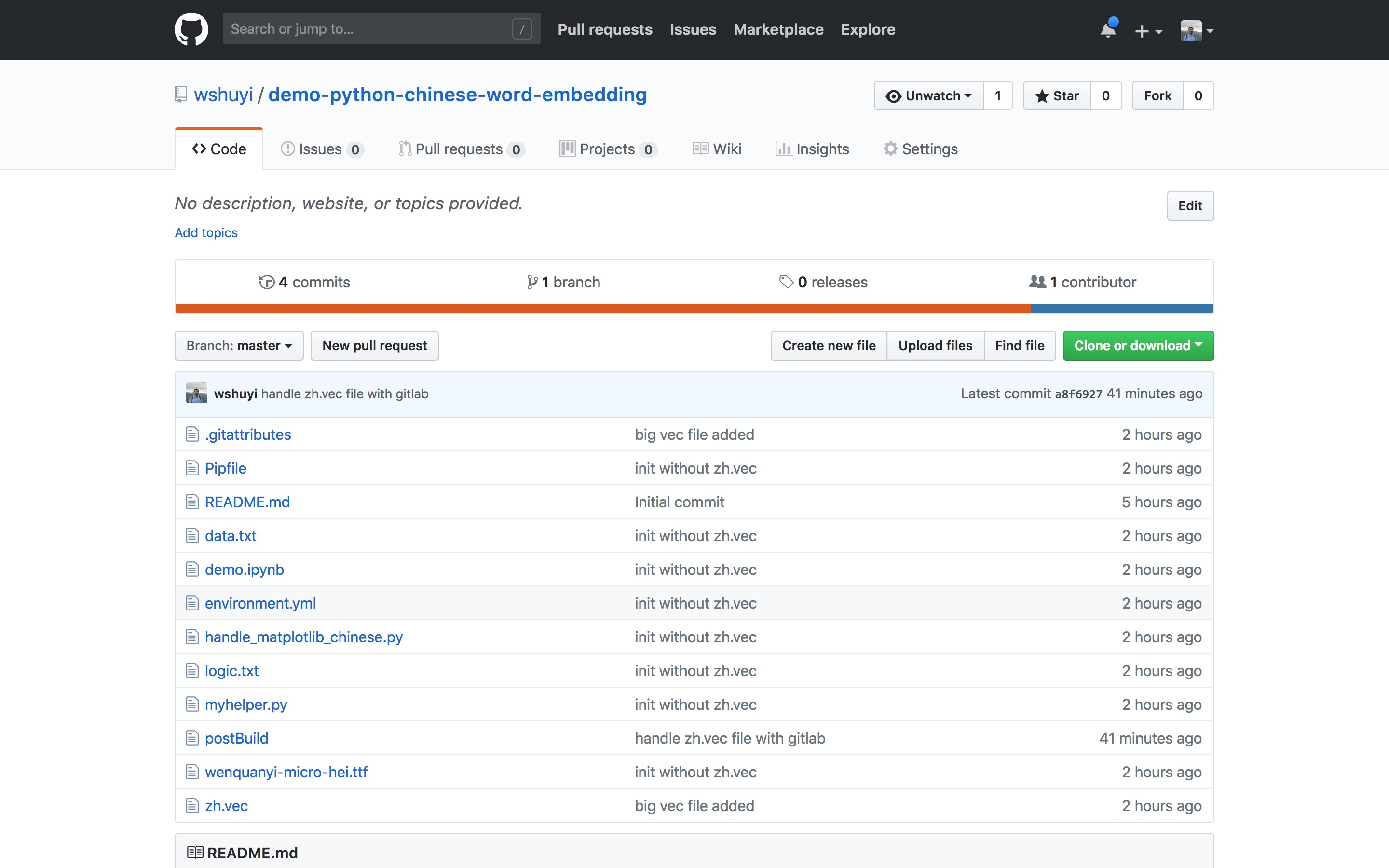### 5.6.4 讲解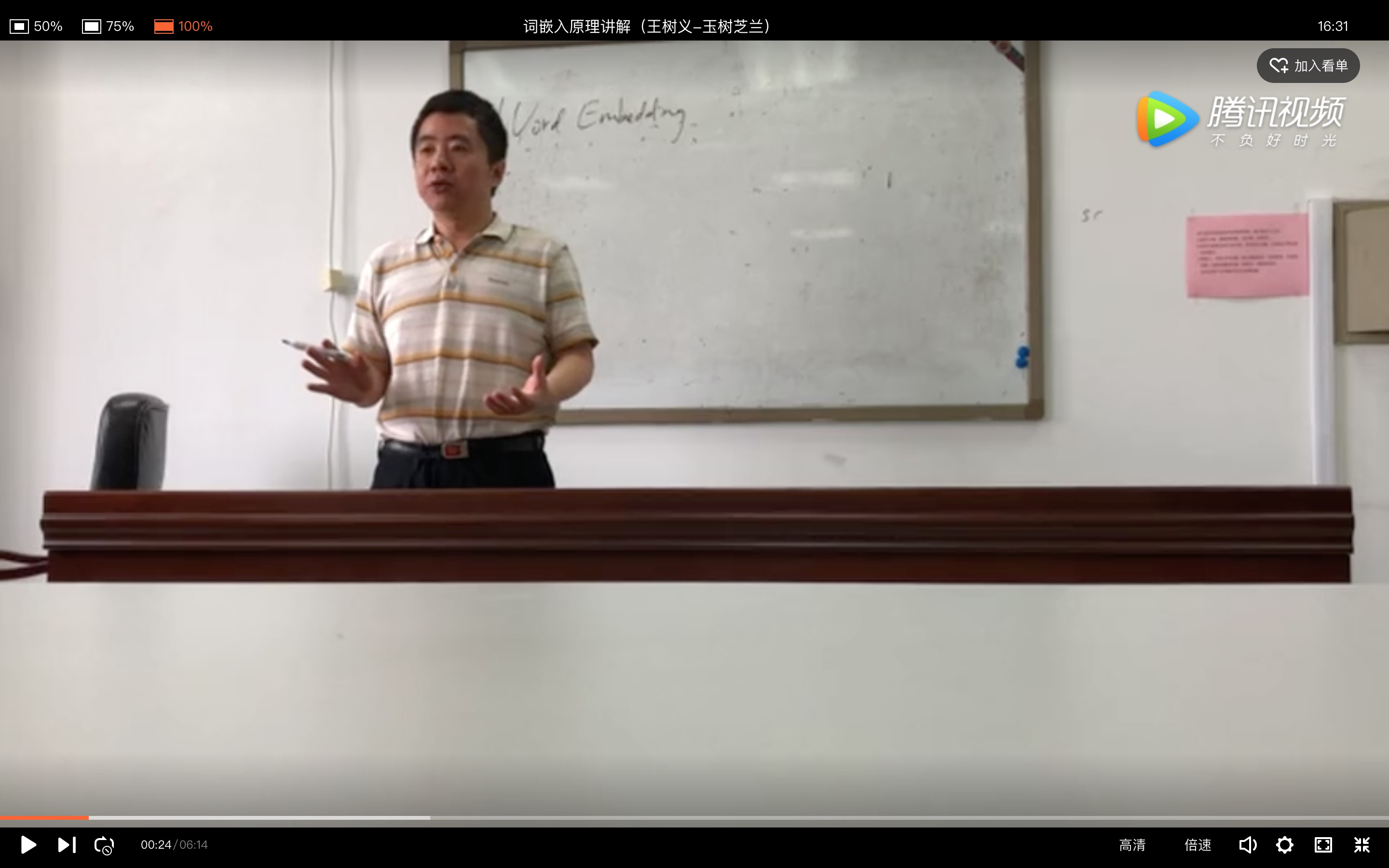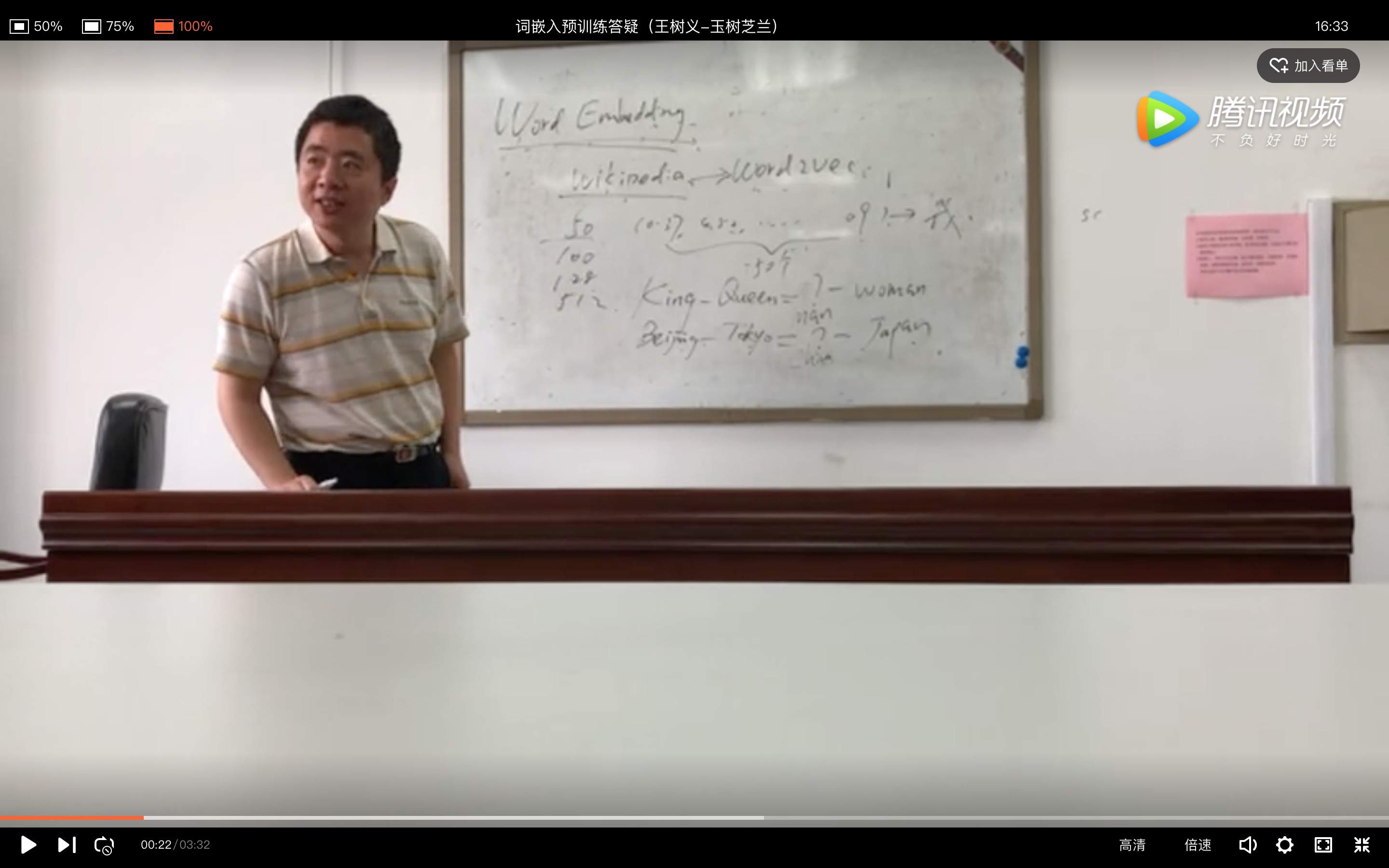## 5.7 本章小结